Search datasheet (1.687.043 components) Search fieldPart namePart descriptionAbout site Manufacturers list ChipFindIC search engine AllXrefCross-reference database### Datasheet: L4931ABDT27 (STMicroelectronics)

VERY LOW DROP VOLTAGE REGULATORS WITH INHIBIT
Download:PDFZIP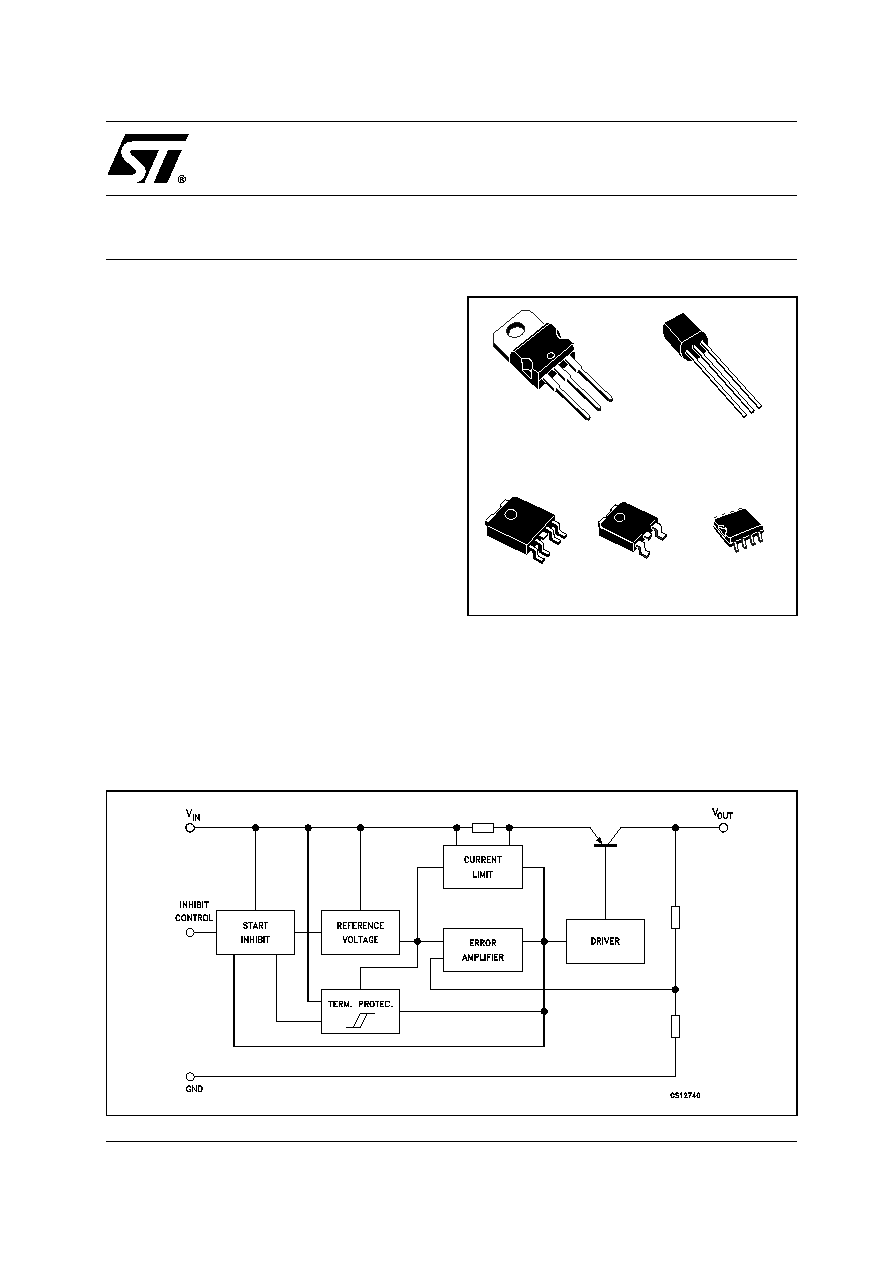1/29
January 2004
s
VERY LOW DROPOUT VOLTAGE (0.4V)
s
VERY LOW QUIESCENT CURRENT
(TYP. 50 µA IN OFF MODE, 600µA IN ON
MODE)
s
OUTPUT CURRENT UP TO 250 mA
s
LOGIC-CONTROLLED ELECTRONIC
SHUTDOWN
s
OUTPUT VOLTAGES OF 1.25; 1.5; 2.5; 2.7;
3; 3.3; 3.5; 4; 4.5; 4.7; 5; 5.2; 5.5; 6; 8; 12V
s
INTERNAL CURRENT AND THERMAL LIMIT
s
ONLY 2.2µF FOR STABILITY
s
AVAILABLE IN ± 1% (AB) OR 2% (C)
SELECTION AT 25°C
s
SUPPLY VOLTAGE REJECTION: 70db TYP.
FOR 5V VERSION
s
TEMPERATURE RANGE: -40 TO 125°C
DESCRIPTION
The L4931 series are very Low Drop regulators
available in TO-220, SO-8, DPAK, PPAK and
TO-92 packages and in a wide range of output
voltages.
The very Low Drop voltage (0.4V) and the very
low quiescent current make them particularly
suitable for Low Noise, Low Power applications
and specially in battery powered systems.
In PPAK and SO-8 packages a Shutdown Logic
Control function is available TTL compatible. This
means that when the device is used as a local
regulator, it is possible to put a part of the board in
standby, decreasing the total power consumption.
It requires only a 2.2 µF capacitor for stability
allowing space and cost saving.
L4931
SERIES
VERY LOW DROP
VOLTAGE REGULATORS WITH INHIBIT
SCHEMATIC DIAGRAM
TO-220
SO-8
TO-92
DPAK
PPAK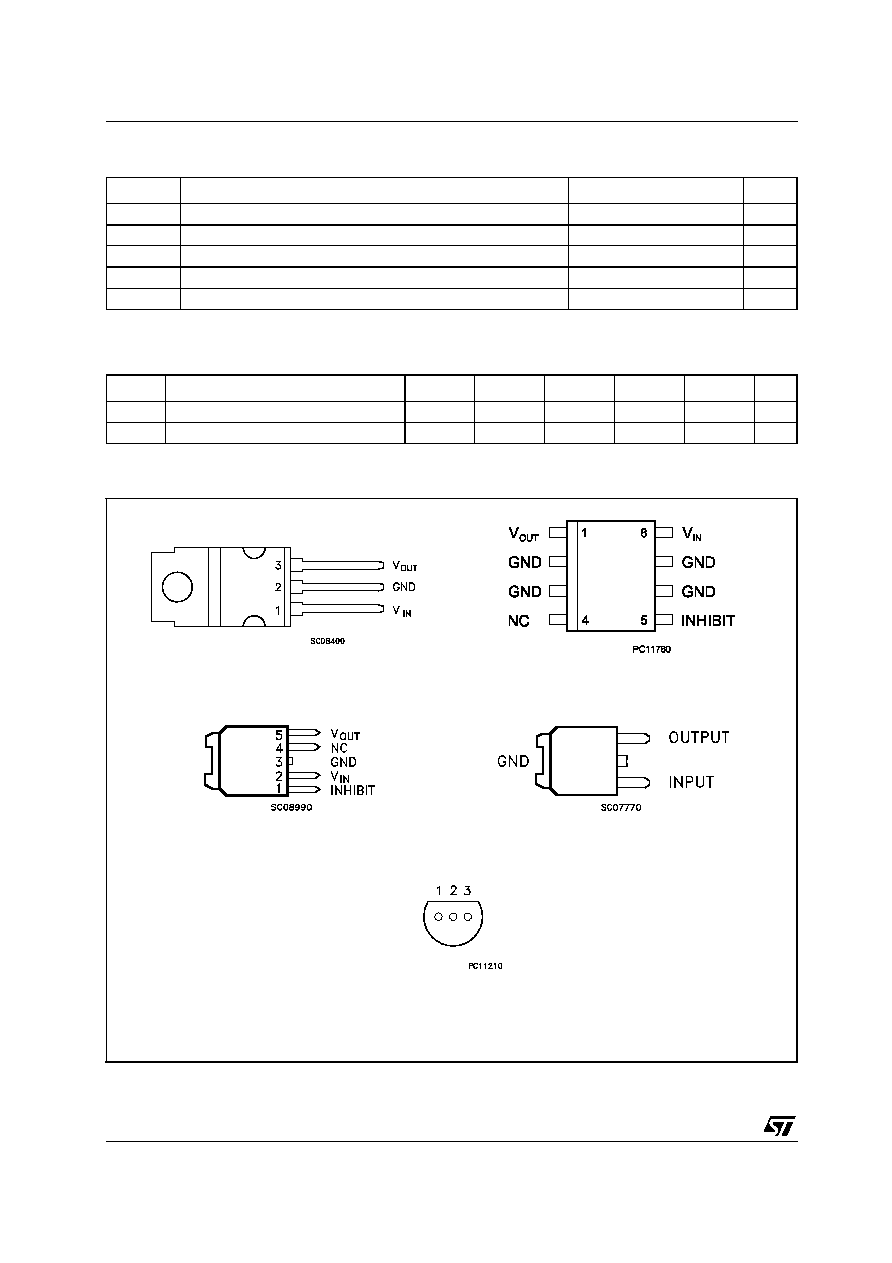L4931 SERIES
2/29
ABSOLUTE MAXIMUM RATINGS
Absolute Maximum Ratings are those values beyond which damage to the device may occur. Functional operation under these condition is
not implied.
THERMAL DATA
(*) Considering 6 cm
2
of copper board heat-sink
CONNECTION DIAGRAM (top view)
Symbol
Parameter
Value
Unit
V
I
DC Input Voltage
20
V
I
O
Output Current
Internally Limited
P
tot
Power Dissipation
Internally Limited
T
stg
Storage Temperature Range
-40 to 150
°C
T
op
Operating Junction Temperature Range
-40 to 125
°C
Symbol
Parameter
TO-220
SO-8
DPAK
PPAK
TO-92
Unit
R
thj-case
Thermal Resistance Junction-case
3
20
8
8
°C/W
R
thj-amb
Thermal Resistance Junction-ambient
50
55(*)
100
100
200
°C/W
TO-220
SO-8
DPAK
PIN 1 = V
OUT
PIN 2 = GND
PIN 3 = V
IN
PPAK
BOTTOM VIEW
TO-92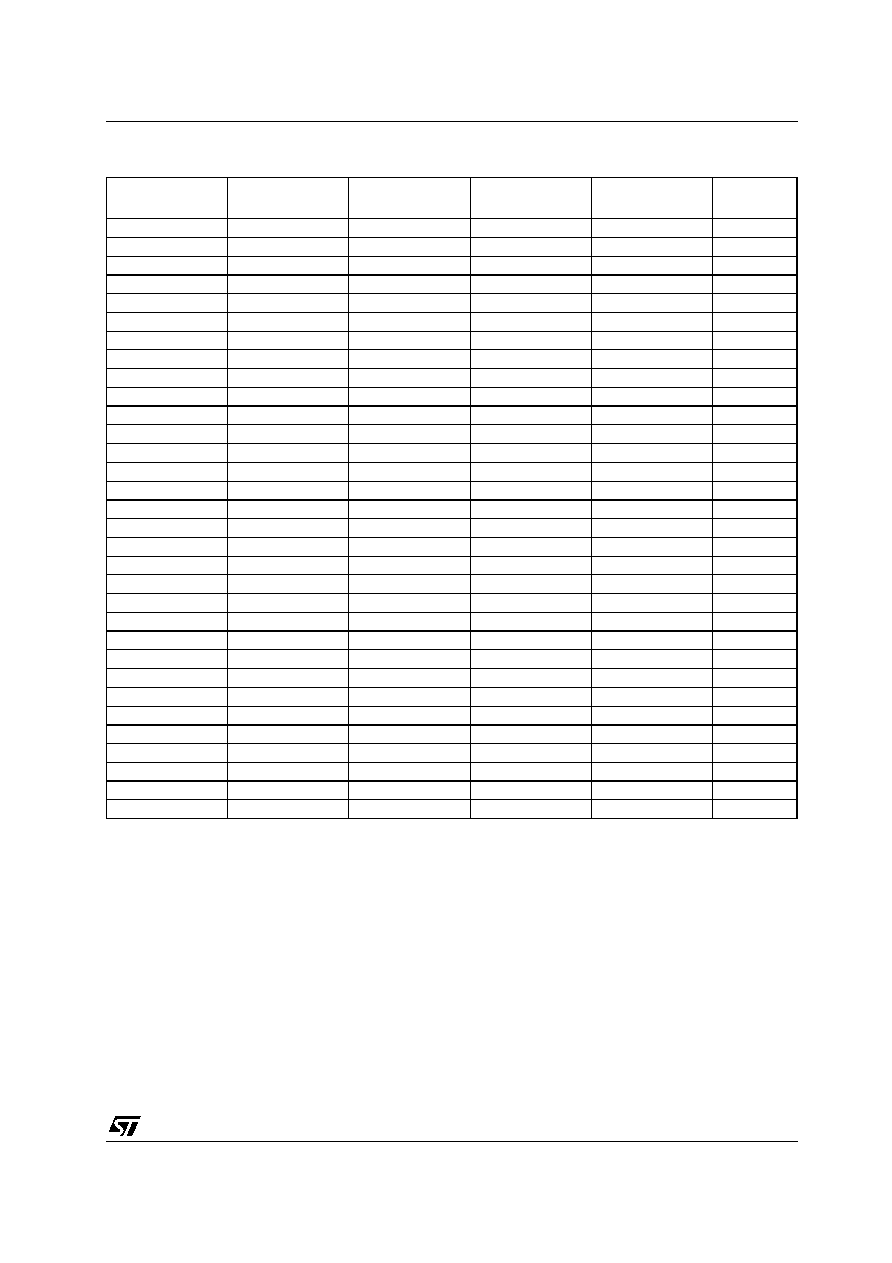L4931 SERIES
3/29
ORDERING CODES
(*) Available on request.
TO-220
SO-8
PPAK
DPAK
TO-92
OUTPUT
VOLTAGE
L4931CV12 (*)
L4931CD12 (*)
L4931CPT12 (*)
L4931CDT12 (*)
L4931CZ12 (*)
1.25 V
L4931ABV12 (*)
L4931ABD12 (*)
L4931ABPT12 (*)
L4931ABDT12 (*)
L4931ABZ12 (*)
1.25 V
L4931CV15 (*)
L4931CD15 (*)
L4931CPT15 (*)
L4931CDT15 (*)
L4931CZ15 (*)
1.5 V
L4931ABV15 (*)
L4931ABD15 (*)
L4931ABPT15 (*)
L4931ABDT15 (*)
L4931ABZ15 (*)
1.5 V
L4931CV25 (*)
L4931CD25
L4931CPT25 (*)
L4931CDT25
L4931CZ25 (*)
2.5 V
L4931ABV25 (*)
L4931ABD25
L4931ABPT25 (*)
L4931ABDT25
L4931ABZ25 (*)
2.5 V
L4931CV27
L4931CD27
L4931CPT27
L4931CDT27
L4931CZ27
2.7 V
L4931ABV27
L4931ABD27
L4931ABPT27
L4931ABDT27
L4931ABZ27
2.7 V
L4931CV30
L4931CD30
L4931CPT30
L4931CDT30
L4931CZ30
3 V
L4931ABV30
L4931ABD30
L4931ABPT30
L4931ABDT30
L4931ABZ30
3 V
L4931CV33
L4931CD33
L4931CPT33
L4931CDT33
L4931CZ33
3.3 V
L4931ABV33
L4931ABD33
L4931ABPT33
L4931ABDT33
L4931ABZ33
3.3 V
L4931CV35
L4931CD35
L4931CPT35
L4931CDT35
L4931CZ35
3.5 V
L4931ABV35
L4931ABD35
L4931ABPT35
L4931ABDT35
L4931ABZ35
3.5 V
L4931CV40
L4931CD40
L4931CPT40
L4931CDT40
L4931CZ40
4 V
L4931ABV40
L4931ABD40
L4931ABPT40
L4931ABDT40
L4931ABZ40
4 V
L4931CV45 (*)
L4931CD45 (*)
L4931CPT45 (*)
L4931CDT45 (*)
L4931CZ45 (*)
4.5 V
L4931ABV45 (*)
L4931ABD45 (*)
L4931ABPT45 (*)
L4931ABDT45 (*)
L4931ABZ45 (*)
4.5 V
L4931CV47
L4931CD47
L4931CPT47
L4931CDT47
L4931CZ47
4.75 V
L4931ABV47
L4931ABD47
L4931ABPT47
L4931ABDT47
L4931ABZ47
4.75 V
L4931CV50
L4931CD50
L4931CPT50
L4931CDT50
L4931CZ50
5 V
L4931ABV50
L4931ABD50
L4931ABPT50
L4931ABDT50
L4931ABZ50
5 V
L4931CV52 (*)
L4931CD52 (*)
L4931CPT52 (*)
L4931CDT52 (*)
L4931CZ52 (*)
5.2 V
L4931ABV52 (*)
L4931ABD52 (*)
L4931ABPT52 (*)
L4931ABDT52 (*)
L4931ABZ52 (*)
5.2 V
L4931CV55 (*)
L4931CD55 (*)
L4931CPT55 (*)
L4931CDT55 (*)
L4931CZ55 (*)
5.5 V
L4931ABV55 (*)
L4931ABD55 (*)
L4931ABPT55 (*)
L4931ABDT55 (*)
L4931ABZ55 (*)
5.5 V
L4931CV60
L4931CD60
L4931CPT60
L4931CDT60
L4931CZ60
6 V
L4931ABV60
L4931ABD60
L4931ABPT60
L4931ABDT60
L4931ABZ60
6 V
L4931CV80
L4931CD80
L4931CPT80
L4931CDT80
L4931CZ80
8 V
L4931ABV80
L4931ABD80
L4931ABPT80
L4931ABDT80
L4931ABZ80
8 V
L4931CV120
L4931CD120
L4931CPT120
L4931CDT120
L4931CZ120
12 V
L4931ABV120
L4931ABD120
L4931ABPT120
L4931ABDT120
L4931ABZ120
12 V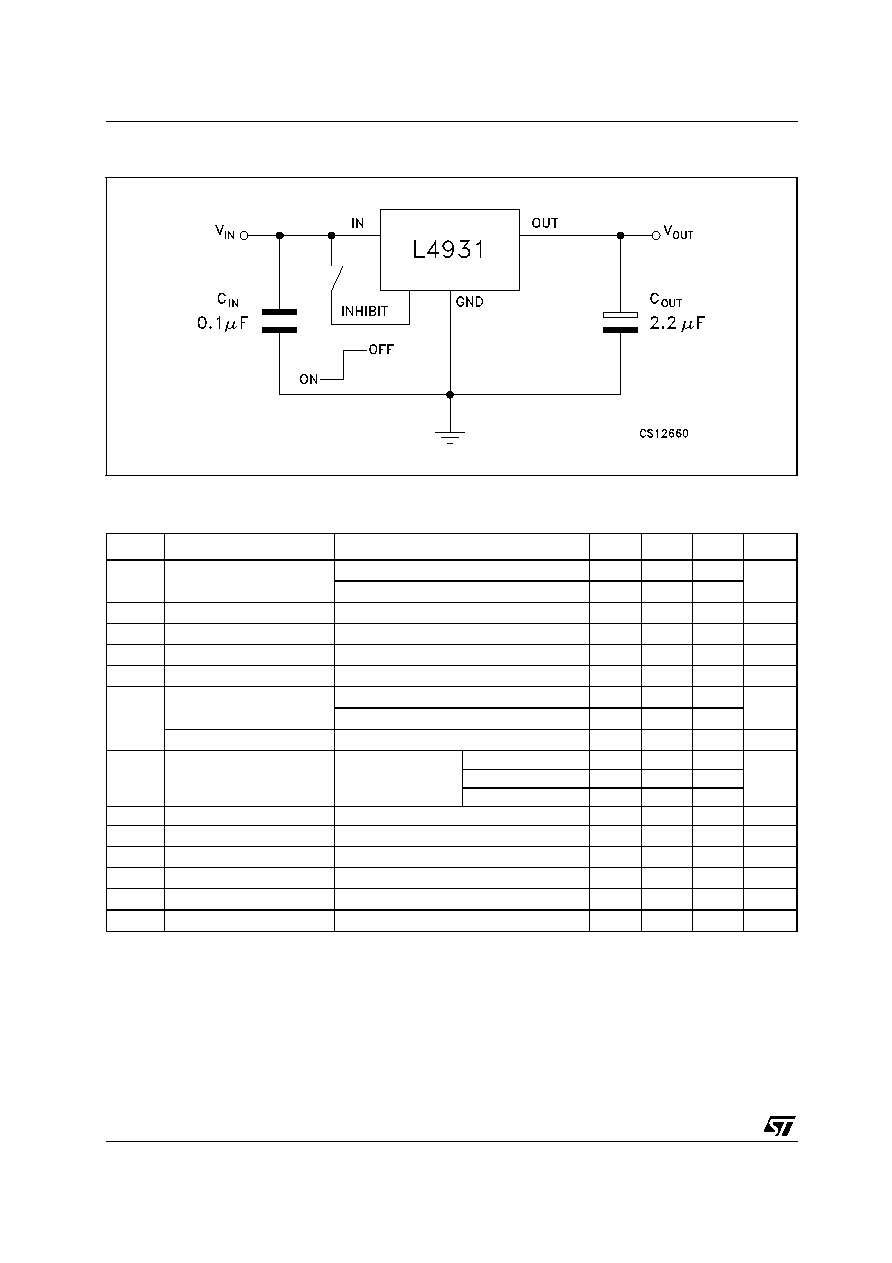L4931 SERIES
4/29
TEST CIRCUITS
ELECTRICAL CHARACTERISTICS OF L4931ABxx12 (refer to the test circuits, T
J
= 25°C,
C
I
= 0.1 µF, C
O
= 2.2 µF unless otherwise specified).
(*) For SO-8 package the maximum limit of load regulation and dropout is increased by 20 mV.
Symbol
Parameter
Test Conditions
Min.
Typ.
Max.
Unit
V
O
Output Voltage
I
O
= 5 mA
V
I
= 3.3 V
1.238
1.25
1.263
V
I
O
= 5 mA
V
I
= 3.3 V
T
A
=-25 to 85°C
1.225
1.275
V
I
Operating Input Voltage
I
O
= 250 mA
2.5
20
V
I
out
Output Current Limit
300
mA
V
O
Line Regulation
V
I
= 2.5 to 20 V
I
O
= 0.5 mA
3
15
mV
V
O
(*)
Load Regulation
V
I
= 2.7 V
I
O
= 0.5 to 250 mA
3
15
mV
I
d
Quiescent Current
ON MODE
V
I
= 2.7 to 20 V
I
O
= 0 mA
0.6
1
mA
V
I
= 2.7 to 20 V
I
O
= 250 mA
4
6
OFF MODE
V
I
= 6 V
50
100
µA
SVR
Supply Voltage Rejection
I
O
= 5 mA
V
I
= 3.7 ± 1 V
f = 120 Hz
80
dB
f = 1 KHz
77
f = 10 KHz
55
eN
Output Noise Voltage
B =10 Hz to 100 KHz
50
µ
V
V
d
(*)
Dropout Voltage
I
O
= 250 mA
1.25
V
V
IL
Control Input Logic Low
T
A
= -40 to 125°C
0.8
V
V
IH
Control Input Logic High
T
A
= -40 to 125°C
2
V
I
I
Control Input Current
V
I
= 6 V,
V
C
= 6 V
10
µA
C
O
Output Bypass Capacitance ESR = 0.1 to 10
I
O
= 0 to 250 mA
2
10
µF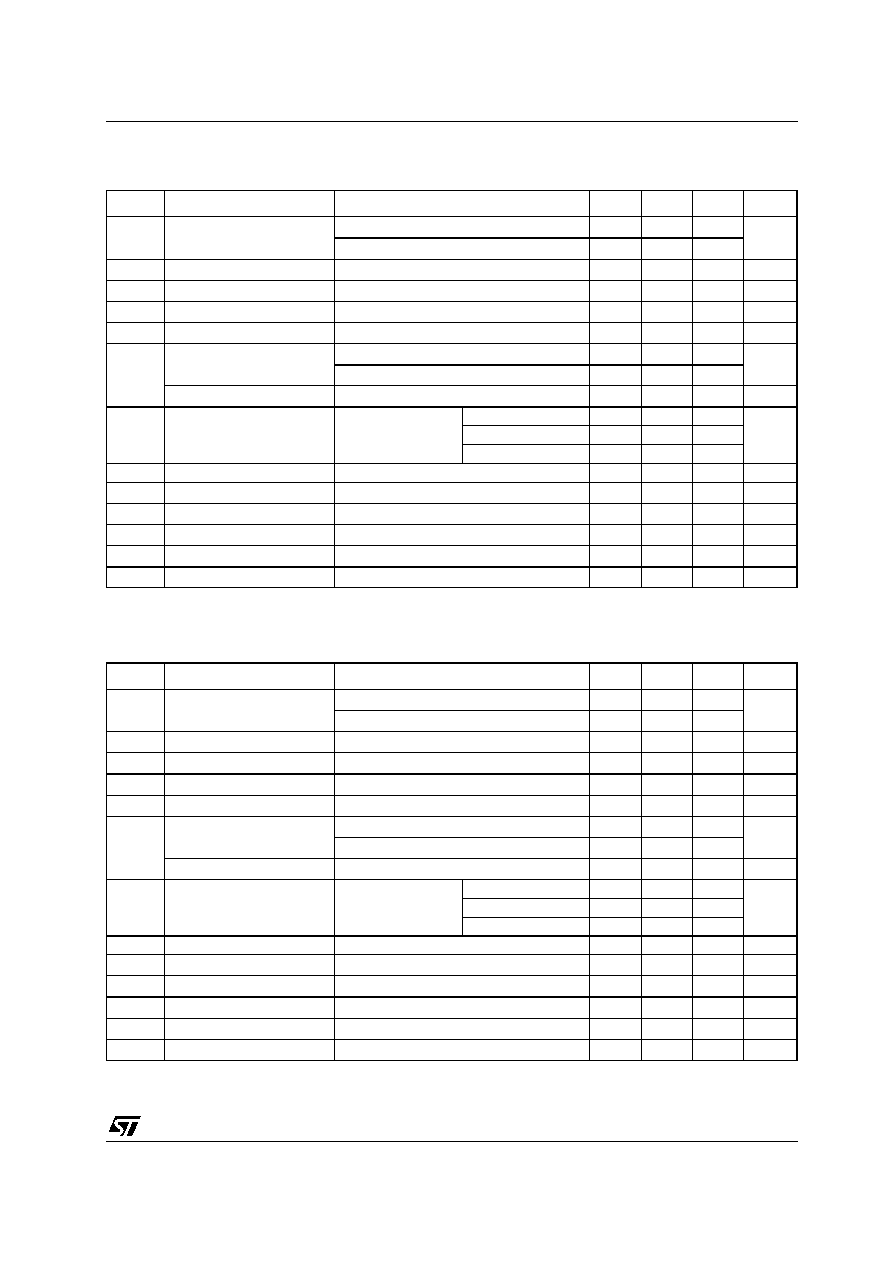L4931 SERIES
5/29
ELECTRICAL CHARACTERISTICS OF L4931Cxx12 (refer to the test circuits, T
J
= 25°C,
C
I
= 0.1 µF, C
O
= 2.2 µF unless otherwise specified).
(*) For SO-8 package the maximum limit of load regulation and dropout is increased by 20 mV.
ELECTRICAL CHARACTERISTICS OF L4931ABxx15 (refer to the test circuits, T
J
= 25°C,
C
I
= 0.1 µF, C
O
= 2.2 µF unless otherwise specified).
(*) For SO-8 package the maximum limit of load regulation and dropout is increased by 20 mV.
Symbol
Parameter
Test Conditions
Min.
Typ.
Max.
Unit
V
O
Output Voltage
I
O
= 5 mA
V
I
= 3.3 V
1.225
1.25
1.275
V
I
O
= 5 mA
V
I
= 3.3 V
T
A
=-25 to 85°C
1.2
1.3
V
I
Operating Input Voltage
I
O
= 250 mA
2.5
20
V
I
out
Output Current Limit
300
mA
V
O
Line Regulation
V
I
= 2.5 to 20 V
I
O
= 0.5 mA
3
18
mV
V
O
(*)
Load Regulation
V
I
= 2.7 V
I
O
= 0.5 to 250 mA
3
18
mV
I
d
Quiescent Current
ON MODE
V
I
= 2.7 to 20 V
I
O
= 0 mA
0.6
1
mA
V
I
= 2.7 to 20 V
I
O
= 250 mA
4
6
OFF MODE
V
I
= 6 V
50
100
µA
SVR
Supply Voltage Rejection
I
O
= 5 mA
V
I
= 3.7 ± 1 V
f = 120 Hz
80
dB
f = 1 KHz
77
f = 10 KHz
55
eN
Output Noise Voltage
B =10 Hz to 100 KHz
50
µ
V
V
d
(*)
Dropout Voltage
I
O
= 250 mA
1.25
V
V
IL
Control Input Logic Low
T
A
= -40 to 125°C
0.8
V
V
IH
Control Input Logic High
T
A
= -40 to 125°C
2
V
I
I
Control Input Current
V
I
= 6 V,
V
C
= 6 V
10
µA
C
O
Output Bypass Capacitance ESR = 0.1 to 10
I
O
= 0 to 250 mA
2
10
µF
Symbol
Parameter
Test Conditions
Min.
Typ.
Max.
Unit
V
O
Output Voltage
I
O
= 5 mA
V
I
= 3.5 V
1.485
1.5
1.515
V
I
O
= 5 mA
V
I
= 3.5 V
T
A
=-25 to 85°C
1.47
1.53
V
I
Operating Input Voltage
I
O
= 250 mA
2.5
20
V
I
out
Output Current Limit
300
mA
V
O
Line Regulation
V
I
= 2.5 to 20 V
I
O
= 0.5 mA
3
15
mV
V
O
(*)
Load Regulation
V
I
= 2.7 V
I
O
= 0.5 to 250 mA
3
15
mV
I
d
Quiescent Current
ON MODE
V
I
= 2.7 to 20 V
I
O
= 0 mA
0.6
1
mA
V
I
= 2.7 to 20 V
I
O
= 250 mA
4
6
OFF MODE
V
I
= 6 V
50
100
µA
SVR
Supply Voltage Rejection
I
O
= 5 mA
V
I
= 3.7 ± 1 V
f = 120 Hz
79
dB
f = 1 KHz
76
f = 10 KHz
55
eN
Output Noise Voltage
B =10 Hz to 100 KHz
50
µ
V
V
d
(*)
Dropout Voltage
I
O
= 250 mA
1
V
V
IL
Control Input Logic Low
T
A
= -40 to 125°C
0.8
V
V
IH
Control Input Logic High
T
A
= -40 to 125°C
2
V
I
I
Control Input Current
V
I
= 6 V,
V
C
= 6 V
10
µA
C
O
Output Bypass Capacitance ESR = 0.1 to 10
I
O
= 0 to 250 mA
2
10
µF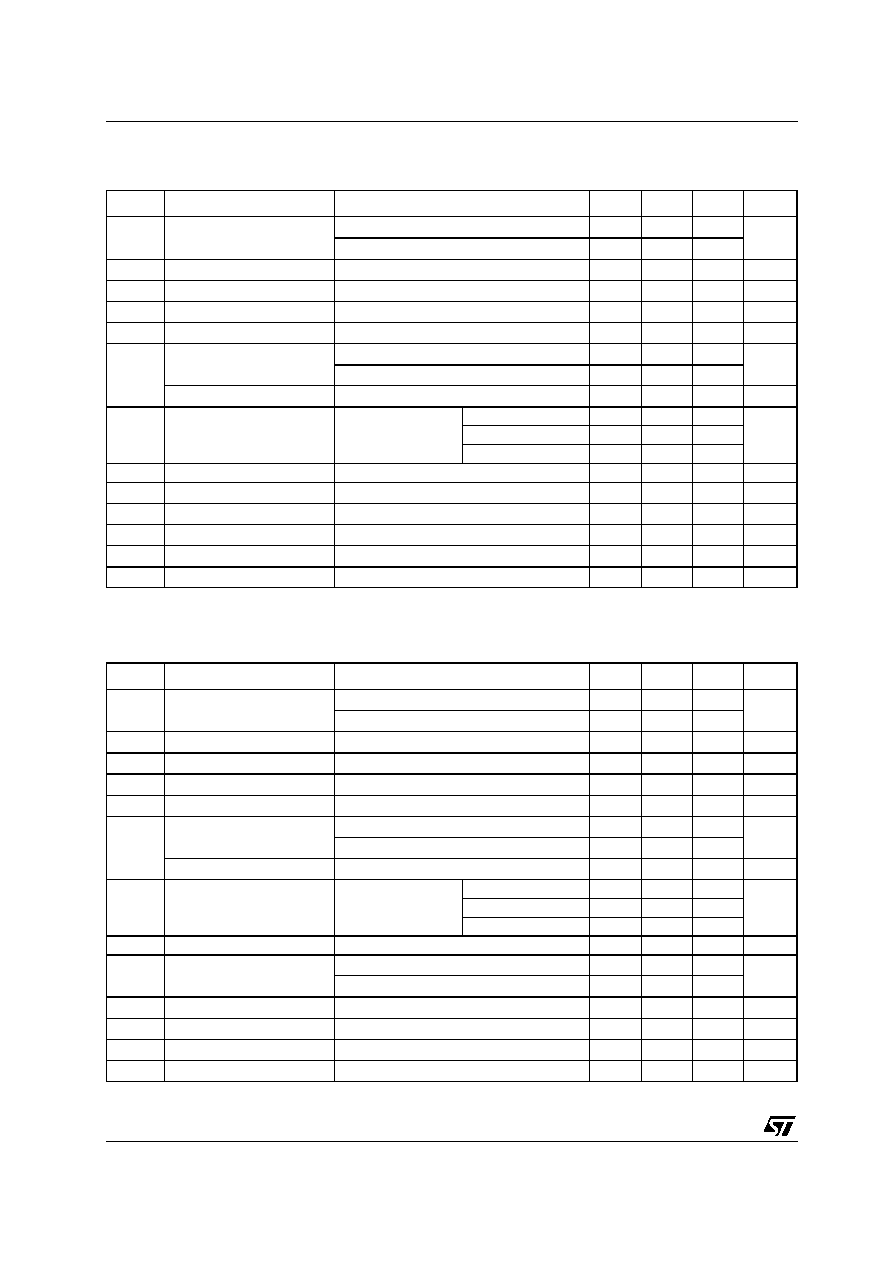L4931 SERIES
6/29
ELECTRICAL CHARACTERISTICS OF L4931Cxx15 (refer to the test circuits, T
J
= 25°C,
C
I
= 0.1 µF, C
O
= 2.2 µF unless otherwise specified).
(*) For SO-8 package the maximum limit of load regulation and dropout is increased by 20 mV.
ELECTRICAL CHARACTERISTICS OF L4931ABxx25 (refer to the test circuits, T
J
= 25°C,
C
I
= 0.1 µF, C
O
= 2.2 µF unless otherwise specified).
(*) For SO-8 package the maximum limit of load regulation and dropout is increased by 20 mV.
Symbol
Parameter
Test Conditions
Min.
Typ.
Max.
Unit
V
O
Output Voltage
I
O
= 5 mA
V
I
= 3.5 V
1.47
1.5
1.53
V
I
O
= 5 mA
V
I
= 3.5 V
T
A
=-25 to 85°C
1.44
1.56
V
I
Operating Input Voltage
I
O
= 250 mA
2.5
20
V
I
out
Output Current Limit
300
mA
V
O
Line Regulation
V
I
= 2.5 to 20 V
I
O
= 0.5 mA
3
18
mV
V
O
(*)
Load Regulation
V
I
= 2.7 V
I
O
= 0.5 to 250 mA
3
18
mV
I
d
Quiescent Current
ON MODE
V
I
= 2.7 to 20 V
I
O
= 0 mA
0.6
1
mA
V
I
= 2.7 to 20 V
I
O
= 250 mA
4
6
OFF MODE
V
I
= 6 V
50
100
µA
SVR
Supply Voltage Rejection
I
O
= 5 mA
V
I
= 3.7 ± 1 V
f = 120 Hz
79
dB
f = 1 KHz
76
f = 10 KHz
55
eN
Output Noise Voltage
B =10 Hz to 100 KHz
50
µ
V
V
d
(*)
Dropout Voltage
I
O
= 250 mA
1
V
V
IL
Control Input Logic Low
T
A
= -40 to 125°C
0.8
V
V
IH
Control Input Logic High
T
A
= -40 to 125°C
2
V
I
I
Control Input Current
V
I
= 6 V,
V
C
= 6 V
10
µA
C
O
Output Bypass Capacitance ESR = 0.1 to 10
I
O
= 0 to 250 mA
2
10
µF
Symbol
Parameter
Test Conditions
Min.
Typ.
Max.
Unit
V
O
Output Voltage
I
O
= 5 mA
V
I
= 4.5 V
2.475
2.5
2.525
V
I
O
= 5 mA
V
I
= 4.5 V
T
A
=-25 to 85°C
2.45
2.55
V
I
Operating Input Voltage
I
O
= 250 mA
20
V
I
out
Output Current Limit
300
mA
V
O
Line Regulation
V
I
= 3.2 to 20 V
I
O
= 0.5 mA
3
15
mV
V
O
(*)
Load Regulation
V
I
= 3.4 V
I
O
= 0.5 to 250 mA
3
15
mV
I
d
Quiescent Current
ON MODE
V
I
= 3.4 to 20 V
I
O
= 0 mA
0.6
1
mA
V
I
= 3.4 to 20 V
I
O
= 250 mA
4
6
OFF MODE
V
I
= 6 V
50
100
µA
SVR
Supply Voltage Rejection
I
O
= 5 mA
V
I
= 4.4 ± 1 V
f = 120 Hz
75
dB
f = 1 KHz
72
f = 10 KHz
55
eN
Output Noise Voltage
B =10 Hz to 100 KHz
50
µ
V
V
d
(*)
Dropout Voltage
I
O
= 250 mA
0.4
0.6
V
I
O
= 250 mA
T
J
= -40 to 125°C
0.8
V
IL
Control Input Logic Low
T
A
= -40 to 125°C
0.8
V
V
IH
Control Input Logic High
T
A
= -40 to 125°C
2
V
I
I
Control Input Current
V
I
= 6 V,
V
C
= 6 V
10
µA
C
O
Output Bypass Capacitance ESR = 0.1 to 10
I
O
= 0 to 250 mA
2
10
µF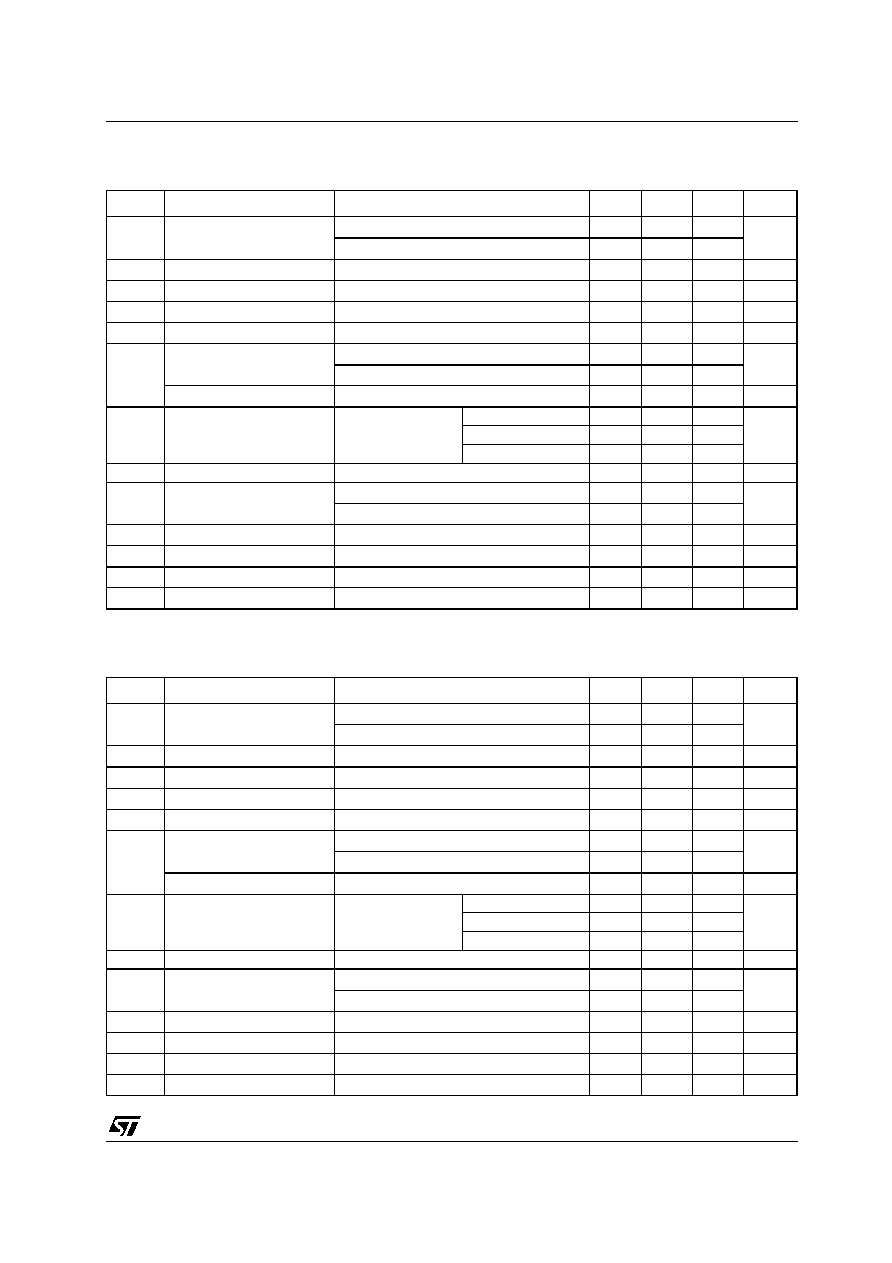L4931 SERIES
7/29
ELECTRICAL CHARACTERISTICS OF L4931Cxx25 (refer to the test circuits, T
J
= 25°C,
C
I
= 0.1 µF, C
O
= 2.2 µF unless otherwise specified).
(*) For SO-8 package the maximum limit of load regulation and dropout is increased by 20 mV.
ELECTRICAL CHARACTERISTICS OF L4931ABxx27 (refer to the test circuits, T
J
= 25°C,
C
I
= 0.1 µF, C
O
= 2.2 µF unless otherwise specified).
(*) For SO-8 package the maximum limit of load regulation and dropout is increased by 20 mV.
Symbol
Parameter
Test Conditions
Min.
Typ.
Max.
Unit
V
O
Output Voltage
I
O
= 5 mA
V
I
= 4.5 V
2.45
2.5
2.55
V
I
O
= 5 mA
V
I
= 4.5 V
T
A
=-25 to 85°C
2.4
2.6
V
I
Operating Input Voltage
I
O
= 250 mA
20
V
I
out
Output Current Limit
300
mA
V
O
Line Regulation
V
I
= 3.3 to 20 V
I
O
= 0.5 mA
3
18
mV
V
O
(*)
Load Regulation
V
I
= 3.5 V
I
O
= 0.5 to 250 mA
3
18
mV
I
d
Quiescent Current
ON MODE
V
I
= 3.5 to 20 V
I
O
= 0 mA
0.6
1
mA
V
I
= 3.5 to 20 V
I
O
= 250 mA
4
6
OFF MODE
V
I
= 6 V
50
100
µA
SVR
Supply Voltage Rejection
I
O
= 5 mA
V
I
= 4.4 ± 1 V
f = 120 Hz
75
dB
f = 1 KHz
72
f = 10 KHz
55
eN
Output Noise Voltage
B =10 Hz to 100 KHz
50
µ
V
V
d
(*)
Dropout Voltage
I
O
= 250 mA
0.4
0.6
V
I
O
= 250 mA
T
J
= -40 to 125°C
0.8
V
IL
Control Input Logic Low
T
A
= -40 to 125°C
0.8
V
V
IH
Control Input Logic High
T
A
= -40 to 125°C
2
V
I
I
Control Input Current
V
I
= 6 V,
V
C
= 6 V
10
µA
C
O
Output Bypass Capacitance ESR = 0.1 to 10
I
O
= 0 to 250 mA
2
10
µF
Symbol
Parameter
Test Conditions
Min.
Typ.
Max.
Unit
V
O
Output Voltage
I
O
= 5 mA
V
I
= 4.7 V
2.673
2.7
2.727
V
I
O
= 5 mA
V
I
= 4.7 V
T
A
=-25 to 85°C
2.646
2.754
V
I
Operating Input Voltage
I
O
= 250 mA
20
V
I
out
Output Current Limit
300
mA
V
O
Line Regulation
V
I
= 3.4 to 20 V
I
O
= 0.5 mA
3
15
mV
V
O
(*)
Load Regulation
V
I
= 3.6 V
I
O
= 0.5 to 250 mA
3
15
mV
I
d
Quiescent Current
ON MODE
V
I
= 3.6 to 20 V
I
O
= 0 mA
0.6
1
mA
V
I
= 3.6 to 20 V
I
O
= 250 mA
4
6
OFF MODE
V
I
= 6 V
50
100
µA
SVR
Supply Voltage Rejection
I
O
= 5 mA
V
I
= 4.6 ± 1 V
f = 120 Hz
74
dB
f = 1 KHz
71
f = 10 KHz
55
eN
Output Noise Voltage
B =10 Hz to 100 KHz
50
µ
V
V
d
(*)
Dropout Voltage
I
O
= 250 mA
0.4
0.6
V
I
O
= 250 mA
T
J
= -40 to 125°C
0.8
V
IL
Control Input Logic Low
T
A
= -40 to 125°C
0.8
V
V
IH
Control Input Logic High
T
A
= -40 to 125°C
2
V
I
I
Control Input Current
V
I
= 6 V,
V
C
= 6 V
10
µA
C
O
Output Bypass Capacitance ESR = 0.1 to 10
I
O
= 0 to 250 mA
2
10
µF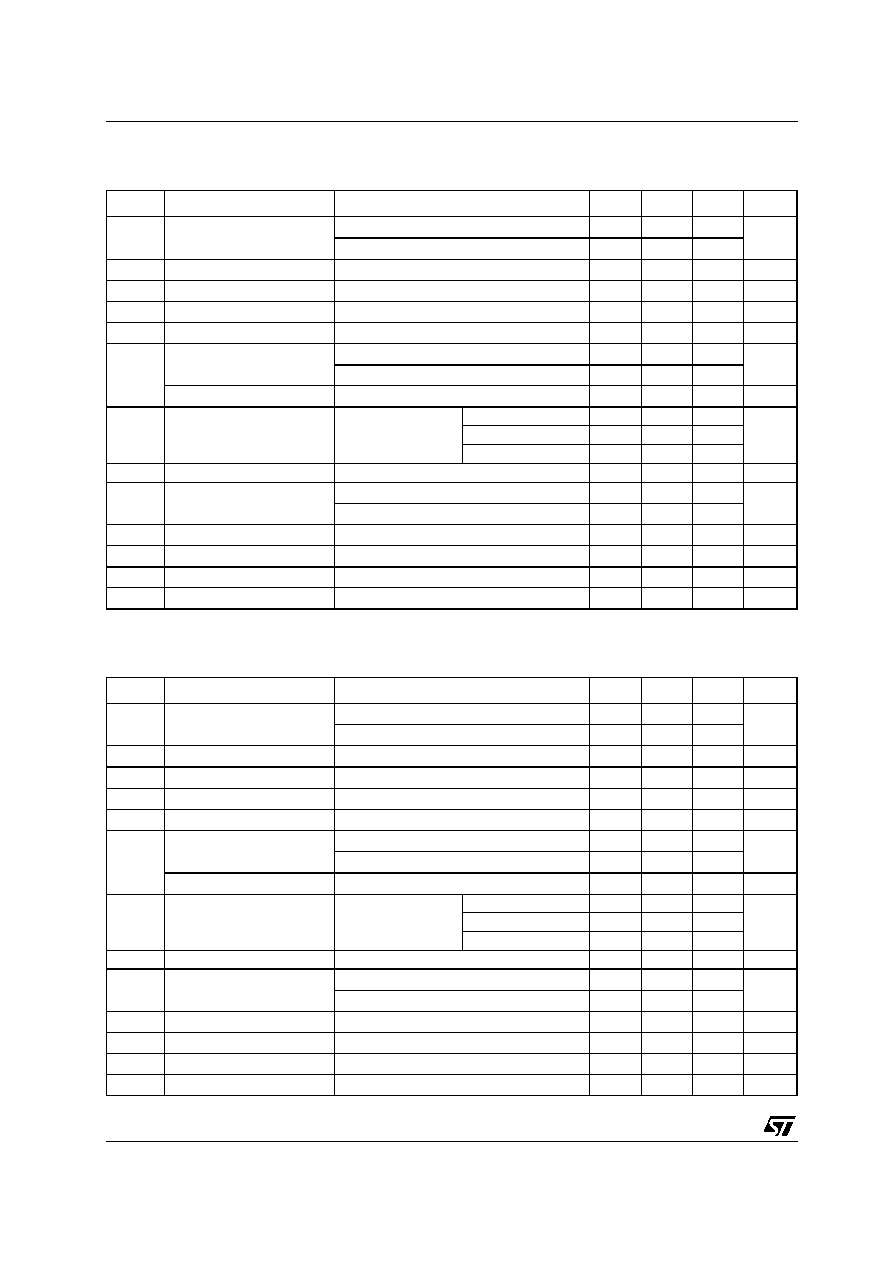L4931 SERIES
8/29
ELECTRICAL CHARACTERISTICS OF L4931Cxx27 (refer to the test circuits, T
J
= 25°C,
C
I
= 0.1 µF, C
O
= 2.2 µF unless otherwise specified).
.
(*) For SO-8 package the maximum limit of load regulation and dropout is increased by 20 mV.
ELECTRICAL CHARACTERISTICS OF L4931ABxx30 (refer to the test circuits, T
J
= 25°C,
C
I
= 0.1 µF, C
O
= 2.2 µF unless otherwise specified).
(*) For SO-8 package the maximum limit of load regulation and dropout is increased by 20 mV.
Symbol
Parameter
Test Conditions
Min.
Typ.
Max.
Unit
V
O
Output Voltage
I
O
= 5 mA
V
I
= 4.7 V
2.646
2.7
2.754
V
I
O
= 5 mA
V
I
= 4.7 V
T
A
=-25 to 85°C
2.592
2.808
V
I
Operating Input Voltage
I
O
= 250 mA
20
V
I
out
Output Current Limit
300
mA
V
O
Line Regulation
V
I
= 3.4 to 20 V
I
O
= 0.5 mA
3
18
mV
V
O
(*)
Load Regulation
V
I
= 3.6 V
I
O
= 0.5 to 250 mA
3
18
mV
I
d
Quiescent Current
ON MODE
V
I
= 3.6 to 20 V
I
O
= 0 mA
0.6
1
mA
V
I
= 3.6 to 20 V
I
O
= 250 mA
4
6
OFF MODE
V
I
= 6 V
50
100
µA
SVR
Supply Voltage Rejection
I
O
= 5 mA
V
I
= 4.6 ± 1 V
f = 120 Hz
74
dB
f = 1 KHz
71
f = 10 KHz
55
eN
Output Noise Voltage
B =10 Hz to 100 KHz
50
µ
V
V
d
(*)
Dropout Voltage
I
O
= 250 mA
0.4
0.6
V
I
O
= 250 mA
T
J
= -40 to 125°C
0.8
V
IL
Control Input Logic Low
T
A
= -40 to 125°C
0.8
V
V
IH
Control Input Logic High
T
A
= -40 to 125°C
2
V
I
I
Control Input Current
V
I
= 6 V,
V
C
= 6 V
10
µA
C
O
Output Bypass Capacitance ESR = 0.1 to 10
I
O
= 0 to 250 mA
2
10
µF
Symbol
Parameter
Test Conditions
Min.
Typ.
Max.
Unit
V
O
Output Voltage
I
O
= 5 mA
V
I
= 5 V
2.97
3
3.03
V
I
O
= 5 mA
V
I
= 5 V
T
A
=-25 to 85°C
2.94
3.06
V
I
Operating Input Voltage
I
O
= 250 mA
20
V
I
out
Output Current Limit
300
mA
V
O
Line Regulation
V
I
= 3.7 to 20 V
I
O
= 0.5 mA
3
15
mV
V
O
(*)
Load Regulation
V
I
= 3.9 V
I
O
= 0.5 to 250 mA
3
15
mV
I
d
Quiescent Current
ON MODE
V
I
= 3.9 to 20 V
I
O
= 0 mA
0.6
1
mA
V
I
= 3.9 to 20 V
I
O
= 250 mA
4
6
OFF MODE
V
I
= 6 V
50
100
µA
SVR
Supply Voltage Rejection
I
O
= 5 mA
V
I
= 4.9 ± 1 V
f = 120 Hz
74
dB
f = 1 KHz
71
f = 10 KHz
55
eN
Output Noise Voltage
B =10 Hz to 100 KHz
50
µ
V
V
d
(*)
Dropout Voltage
I
O
= 250 mA
0.4
0.6
V
I
O
= 250 mA
T
J
= -40 to 125°C
0.8
V
IL
Control Input Logic Low
T
A
= -40 to 125°C
0.8
V
V
IH
Control Input Logic High
T
A
= -40 to 125°C
2
V
I
I
Control Input Current
V
I
= 6 V,
V
C
= 6 V
10
µA
C
O
Output Bypass Capacitance ESR = 0.1 to 10
I
O
= 0 to 250 mA
2
10
µFL4931 SERIES
9/29
ELECTRICAL CHARACTERISTICS OF L4931Cxx30 (refer to the test circuits, T
J
= 25°C,
C
I
= 0.1 µF, C
O
= 2.2 µF unless otherwise specified).
(*) For SO-8 package the maximum limit of load regulation and dropout is increased by 20 mV.
ELECTRICAL CHARACTERISTICS OF L4931ABxx33 (refer to the test circuits, T
J
= 25°C,
C
I
= 0.1 µF, C
O
= 2.2 µF unless otherwise specified).
(*) For SO-8 package the maximum limit of load regulation and dropout is increased by 20 mV.
Symbol
Parameter
Test Conditions
Min.
Typ.
Max.
Unit
V
O
Output Voltage
I
O
= 5 mA
V
I
= 5 V
2.94
3
3.06
V
I
O
= 5 mA
V
I
= 5 V
T
A
=-25 to 85°C
2.88
3.12
V
I
Operating Input Voltage
I
O
= 250 mA
20
V
I
out
Output Current Limit
300
mA
V
O
Line Regulation
V
I
= 3.8 to 20 V
I
O
= 0.5 mA
3
18
mV
V
O
(*)
Load Regulation
V
I
= 4 V
I
O
= 0.5 to 250 mA
3
18
mV
I
d
Quiescent Current
ON MODE
V
I
= 4 to 20 V
I
O
= 0 mA
0.6
1
mA
V
I
= 4 to 20 V
I
O
= 250 mA
4
6
OFF MODE
V
I
= 6 V
50
100
µA
SVR
Supply Voltage Rejection
I
O
= 5 mA
V
I
= 5 ± 1 V
f = 120 Hz
74
dB
f = 1 KHz
71
f = 10 KHz
55
eN
Output Noise Voltage
B =10 Hz to 100 KHz
50
µ
V
V
d
(*)
Dropout Voltage
I
O
= 250 mA
0.4
0.6
V
I
O
= 250 mA
T
J
= -40 to 125°C
0.8
V
IL
Control Input Logic Low
T
A
= -40 to 125°C
0.8
V
V
IH
Control Input Logic High
T
A
= -40 to 125°C
2
V
I
I
Control Input Current
V
I
= 6 V,
V
C
= 6 V
10
µA
C
O
Output Bypass Capacitance ESR = 0.1 to 10
I
O
= 0 to 250 mA
2
10
µF
Symbol
Parameter
Test Conditions
Min.
Typ.
Max.
Unit
V
O
Output Voltage
I
O
= 5 mA
V
I
= 5.3 V
3.267
3.3
3.333
V
I
O
= 5 mA
V
I
= 5.3 V
T
A
=-25 to 85°C
3.234
3.366
V
I
Operating Input Voltage
I
O
= 250 mA
20
V
I
out
Output Current Limit
300
mA
V
O
Line Regulation
V
I
= 4 to 20 V
I
O
= 0.5 mA
3
15
mV
V
O
(*)
Load Regulation
V
I
= 4.2 V
I
O
= 0.5 to 250 mA
3
15
mV
I
d
Quiescent Current
ON MODE
V
I
= 4.2 to 20 V
I
O
= 0 mA
0.6
1
mA
V
I
= 4.2 to 20 V
I
O
= 250 mA
4
6
OFF MODE
V
I
= 6 V
50
100
µA
SVR
Supply Voltage Rejection
I
O
= 5 mA
V
I
= 5.2 ± 1 V
f = 120 Hz
73
dB
f = 1 KHz
70
f = 10 KHz
55
eN
Output Noise Voltage
B =10 Hz to 100 KHz
50
µ
V
V
d
(*)
Dropout Voltage
I
O
= 250 mA
0.4
0.6
V
I
O
= 250 mA
T
J
= -40 to 125°C
0.8
V
IL
Control Input Logic Low
T
A
= -40 to 125°C
0.8
V
V
IH
Control Input Logic High
T
A
= -40 to 125°C
2
V
I
I
Control Input Current
V
I
= 6 V,
V
C
= 6 V
10
µA
C
O
Output Bypass Capacitance ESR = 0.1 to 10
I
O
= 0 to 250 mA
2
10
µFL4931 SERIES
10/29
ELECTRICAL CHARACTERISTICS OF L4931Cxx33 (refer to the test circuits, T
J
= 25°C,
C
I
= 0.1 µF, C
O
= 2.2 µF unless otherwise specified).
(*) For SO-8 package the maximum limit of load regulation and dropout is increased by 20 mV.
ELECTRICAL CHARACTERISTICS OF L4931ABxx35 (refer to the test circuits, T
J
= 25°C,
C
I
= 0.1 µF, C
O
= 2.2 µF unless otherwise specified).
(*) For SO-8 package the maximum limit of load regulation and dropout is increased by 20 mV.
Symbol
Parameter
Test Conditions
Min.
Typ.
Max.
Unit
V
O
Output Voltage
I
O
= 5 mA
V
I
= 5.3 V
3.234
3.3
3.366
V
I
O
= 5 mA
V
I
= 5.3 V
T
A
=-25 to 85°C
3.168
3.432
V
I
Operating Input Voltage
I
O
= 250 mA
20
V
I
out
Output Current Limit
300
mA
V
O
Line Regulation
V
I
= 4.1 to 20 V
I
O
= 0.5 mA
3
18
mV
V
O
(*)
Load Regulation
V
I
= 4.3 V
I
O
= 0.5 to 250 mA
3
18
mV
I
d
Quiescent Current
ON MODE
V
I
= 4.3 to 20 V
I
O
= 0 mA
0.6
1
mA
V
I
= 4.3 to 20 V
I
O
= 250 mA
4
6
OFF MODE
V
I
= 6 V
50
100
µA
SVR
Supply Voltage Rejection
I
O
= 5 mA
V
I
= 5.3 ± 1 V
f = 120 Hz
73
dB
f = 1 KHz
70
f = 10 KHz
55
eN
Output Noise Voltage
B =10 Hz to 100 KHz
50
µ
V
V
d
(*)
Dropout Voltage
I
O
= 250 mA
0.4
0.6
V
I
O
= 250 mA
T
J
= -40 to 125°C
0.8
V
IL
Control Input Logic Low
T
A
= -40 to 125°C
0.8
V
V
IH
Control Input Logic High
T
A
= -40 to 125°C
2
V
I
I
Control Input Current
V
I
= 6 V,
V
C
= 6 V
10
µA
C
O
Output Bypass Capacitance ESR = 0.1 to 10
I
O
= 0 to 250 mA
2
10
µF
Symbol
Parameter
Test Conditions
Min.
Typ.
Max.
Unit
V
O
Output Voltage
I
O
= 5 mA
V
I
= 5.5 V
3.465
3.5
3.535
V
I
O
= 5 mA
V
I
= 5.5 V
T
A
=-25 to 85°C
3.43
3.57
V
I
Operating Input Voltage
I
O
= 250 mA
20
V
I
out
Output Current Limit
300
mA
V
O
Line Regulation
V
I
= 4.2 to 20 V
I
O
= 0.5 mA
3
15
mV
V
O
(*)
Load Regulation
V
I
= 4.4 V
I
O
= 0.5 to 250 mA
3
15
mV
I
d
Quiescent Current
ON MODE
V
I
= 4.4 to 20 V
I
O
= 0 mA
0.6
1
mA
V
I
= 4.4 to 20 V
I
O
= 250 mA
4
6
OFF MODE
V
I
= 6 V
50
100
µA
SVR
Supply Voltage Rejection
I
O
= 5 mA
V
I
= 5.4 ± 1 V
f = 120 Hz
73
dB
f = 1 KHz
70
f = 10 KHz
55
eN
Output Noise Voltage
B =10 Hz to 100 KHz
50
µ
V
V
d
(*)
Dropout Voltage
I
O
= 250 mA
0.4
0.6
V
I
O
= 250 mA
T
J
= -40 to 125°C
0.8
V
IL
Control Input Logic Low
T
A
= -40 to 125°C
0.8
V
V
IH
Control Input Logic High
T
A
= -40 to 125°C
2
V
I
I
Control Input Current
V
I
= 6 V,
V
C
= 6 V
10
µA
C
O
Output Bypass Capacitance ESR = 0.1 to 10
I
O
= 0 to 250 mA
2
10
µFL4931 SERIES
11/29
ELECTRICAL CHARACTERISTICS OF L4931Cxx35 (refer to the test circuits, T
J
= 25°C,
C
I
= 0.1 µF, C
O
= 2.2 µF unless otherwise specified).
(*) For SO-8 package the maximum limit of load regulation and dropout is increased by 20 mV.
ELECTRICAL CHARACTERISTICS OF L4931ABxx40 (refer to the test circuits, T
J
= 25°C,
C
I
= 0.1 µF, C
O
= 2.2 µF unless otherwise specified).
(*) For SO-8 package the maximum limit of load regulation and dropout is increased by 20 mV.
Symbol
Parameter
Test Conditions
Min.
Typ.
Max.
Unit
V
O
Output Voltage
I
O
= 5 mA
V
I
= 5.5 V
3.43
3.5
3.57
V
I
O
= 5 mA
V
I
= 5.5 V
T
A
=-25 to 85°C
3.36
3.64
V
I
Operating Input Voltage
I
O
= 250 mA
20
V
I
out
Output Current Limit
300
mA
V
O
Line Regulation
V
I
= 4.3 to 20 V
I
O
= 0.5 mA
3
18
mV
V
O
(*)
Load Regulation
V
I
= 4.5 V
I
O
= 0.5 to 250 mA
3
18
mV
I
d
Quiescent Current
ON MODE
V
I
= 4.5 to 20 V
I
O
= 0 mA
0.6
1
mA
V
I
= 4.5 to 20 V
I
O
= 250 mA
4
6
OFF MODE
V
I
= 6 V
50
100
µA
SVR
Supply Voltage Rejection
I
O
= 5 mA
V
I
= 5.5 ± 1 V
f = 120 Hz
73
dB
f = 1 KHz
70
f = 10 KHz
55
eN
Output Noise Voltage
B =10 Hz to 100 KHz
50
µ
V
V
d
(*)
Dropout Voltage
I
O
= 250 mA
0.4
0.6
V
I
O
= 250 mA
T
J
= -40 to 125°C
0.8
V
IL
Control Input Logic Low
T
A
= -40 to 125°C
0.8
V
V
IH
Control Input Logic High
T
A
= -40 to 125°C
2
V
I
I
Control Input Current
V
I
= 6 V,
V
C
= 6 V
10
µA
C
O
Output Bypass Capacitance ESR = 0.1 to 10
I
O
= 0 to 250 mA
2
10
µF
Symbol
Parameter
Test Conditions
Min.
Typ.
Max.
Unit
V
O
Output Voltage
I
O
= 5 mA
V
I
= 6 V
3.96
4
4.04
V
I
O
= 5 mA
V
I
= 6 V
T
A
=-25 to 85°C
3.92
4.08
V
I
Operating Input Voltage
I
O
= 250 mA
20
V
I
out
Output Current Limit
300
mA
V
O
Line Regulation
V
I
= 4.7 to 20 V
I
O
= 0.5 mA
3.5
17.5
mV
V
O
(*)
Load Regulation
V
I
= 4.9 V
I
O
= 0.5 to 250 mA
3
15
mV
I
d
Quiescent Current
ON MODE
V
I
= 4.9 to 20 V
I
O
= 0 mA
0.6
1
mA
V
I
= 4.9 to 20 V
I
O
= 250 mA
4
6
OFF MODE
V
I
= 6 V
50
100
µA
SVR
Supply Voltage Rejection
I
O
= 5 mA
V
I
= 5.9 ± 1 V
f = 120 Hz
72
dB
f = 1 KHz
69
f = 10 KHz
55
eN
Output Noise Voltage
B =10 Hz to 100 KHz
50
µ
V
V
d
(*)
Dropout Voltage
I
O
= 250 mA
0.4
0.6
V
I
O
= 250 mA
T
J
= -40 to 125°C
0.8
V
IL
Control Input Logic Low
T
A
= -40 to 125°C
0.8
V
V
IH
Control Input Logic High
T
A
= -40 to 125°C
2
V
I
I
Control Input Current
V
I
= 6 V,
V
C
= 6 V
10
µA
C
O
Output Bypass Capacitance ESR = 0.1 to 10
I
O
= 0 to 250 mA
2
10
µFL4931 SERIES
12/29
ELECTRICAL CHARACTERISTICS OF L4931Cxx40 (refer to the test circuits, T
J
= 25°C,
C
I
= 0.1 µF, C
O
= 2.2 µF unless otherwise specified).
(*) For SO-8 package the maximum limit of load regulation and dropout is increased by 20 mV.
ELECTRICAL CHARACTERISTICS OF L4931ABxx45 (refer to the test circuits, T
J
= 25°C,
C
I
= 0.1 µF, C
O
= 2.2 µF unless otherwise specified).
(*) For SO-8 package the maximum limit of load regulation and dropout is increased by 20 mV.
Symbol
Parameter
Test Conditions
Min.
Typ.
Max.
Unit
V
O
Output Voltage
I
O
= 5 mA
V
I
= 6 V
3.92
4
4.08
V
I
O
= 5 mA
V
I
= 6 V
T
A
=-25 to 85°C
3.84
4.16
V
I
Operating Input Voltage
I
O
= 250 mA
20
V
I
out
Output Current Limit
300
mA
V
O
Line Regulation
V
I
= 4.8 to 20 V
I
O
= 0.5 mA
3.5
21
mV
V
O
(*)
Load Regulation
V
I
= 5 V
I
O
= 0.5 to 250 mA
3
18
mV
I
d
Quiescent Current
ON MODE
V
I
= 5 to 20 V
I
O
= 0 mA
0.6
1
mA
V
I
= 5 to 20 V
I
O
= 250 mA
4
6
OFF MODE
V
I
= 6 V
50
100
µA
SVR
Supply Voltage Rejection
I
O
= 5 mA
V
I
= 6 ± 1 V
f = 120 Hz
72
dB
f = 1 KHz
69
f = 10 KHz
55
eN
Output Noise Voltage
B =10 Hz to 100 KHz
50
µ
V
V
d
(*)
Dropout Voltage
I
O
= 250 mA
0.4
0.6
V
I
O
= 250 mA
T
J
= -40 to 125°C
0.8
V
IL
Control Input Logic Low
T
A
= -40 to 125°C
0.8
V
V
IH
Control Input Logic High
T
A
= -40 to 125°C
2
V
I
I
Control Input Current
V
I
= 6 V,
V
C
= 6 V
10
µA
C
O
Output Bypass Capacitance ESR = 0.1 to 10
I
O
= 0 to 250 mA
2
10
µF
Symbol
Parameter
Test Conditions
Min.
Typ.
Max.
Unit
V
O
Output Voltage
I
O
= 5 mA
V
I
= 6.5 V
4.455
4.5
4.545
V
I
O
= 5 mA
V
I
= 6.5 V
T
A
=-25 to 85°C
4.41
4.59
V
I
Operating Input Voltage
I
O
= 250 mA
20
V
I
out
Output Current Limit
300
mA
V
O
Line Regulation
V
I
= 5.2 to 20 V
I
O
= 0.5 mA
3.5
17.5
mV
V
O
(*)
Load Regulation
V
I
= 5.4 V
I
O
= 0.5 to 250 mA
3
15
mV
I
d
Quiescent Current
ON MODE
V
I
= 5.4 to 20 V
I
O
= 0 mA
0.6
1
mA
V
I
= 5.4 to 20 V
I
O
= 250 mA
4
6
OFF MODE
V
I
= 6 V
50
100
µA
SVR
Supply Voltage Rejection
I
O
= 5 mA
V
I
= 6.4 ± 1 V
f = 120 Hz
71
dB
f = 1 KHz
68
f = 10 KHz
55
eN
Output Noise Voltage
B =10 Hz to 100 KHz
50
µ
V
V
d
(*)
Dropout Voltage
I
O
= 250 mA
0.4
0.6
V
I
O
= 250 mA
T
J
= -40 to 125°C
0.8
V
IL
Control Input Logic Low
T
A
= -40 to 125°C
0.8
V
V
IH
Control Input Logic High
T
A
= -40 to 125°C
2
V
I
I
Control Input Current
V
I
= 6 V,
V
C
= 6 V
10
µA
C
O
Output Bypass Capacitance ESR = 0.1 to 10
I
O
= 0 to 250 mA
2
10
µFL4931 SERIES
13/29
ELECTRICAL CHARACTERISTICS OF L4931Cxx45 (refer to the test circuits, T
J
= 25°C,
C
I
= 0.1 µF, C
O
= 2.2 µF unless otherwise specified).
(*) For SO-8 package the maximum limit of load regulation and dropout is increased by 20 mV.
ELECTRICAL CHARACTERISTICS OF L4931ABxx47 (refer to the test circuits, T
J
= 25°C,
C
I
= 0.1 µF, C
O
= 2.2 µF unless otherwise specified).
(*) For SO-8 package the maximum limit of load regulation and dropout is increased by 20 mV.
Symbol
Parameter
Test Conditions
Min.
Typ.
Max.
Unit
V
O
Output Voltage
I
O
= 5 mA
V
I
= 6.5 V
4.41
4.5
4.59
V
I
O
= 5 mA
V
I
= 6.5 V
T
A
=-25 to 85°C
4.32
4.68
V
I
Operating Input Voltage
I
O
= 250 mA
20
V
I
out
Output Current Limit
300
mA
V
O
Line Regulation
V
I
= 5.3 to 20 V
I
O
= 0.5 mA
3.5
21
mV
V
O
(*)
Load Regulation
V
I
= 5.5 V
I
O
= 0.5 to 250 mA
3
18
mV
I
d
Quiescent Current
ON MODE
V
I
= 5.5 to 20 V
I
O
= 0 mA
0.6
1
mA
V
I
= 5.5 to 20 V
I
O
= 250 mA
4
6
OFF MODE
V
I
= 6 V
50
100
µA
SVR
Supply Voltage Rejection
I
O
= 5 mA
V
I
= 6.5 ± 1 V
f = 120 Hz
71
dB
f = 1 KHz
68
f = 10 KHz
55
eN
Output Noise Voltage
B =10 Hz to 100 KHz
50
µ
V
V
d
(*)
Dropout Voltage
I
O
= 250 mA
0.4
0.6
V
I
O
= 250 mA
T
J
= -40 to 125°C
0.8
V
IL
Control Input Logic Low
T
A
= -40 to 125°C
0.8
V
V
IH
Control Input Logic High
T
A
= -40 to 125°C
2
V
I
I
Control Input Current
V
I
= 6 V,
V
C
= 6 V
10
µA
C
O
Output Bypass Capacitance ESR = 0.1 to 10
I
O
= 0 to 250 mA
2
10
µF
Symbol
Parameter
Test Conditions
Min.
Typ.
Max.
Unit
V
O
Output Voltage
I
O
= 5 mA
V
I
= 6.7 V
4.653
4.7
4.747
V
I
O
= 5 mA
V
I
= 6.7 V
T
A
=-25 to 85°C
4.606
4.794
V
I
Operating Input Voltage
I
O
= 250 mA
20
V
I
out
Output Current Limit
300
mA
V
O
Line Regulation
V
I
= 5.4 to 20 V
I
O
= 0.5 mA
3.5
17.5
mV
V
O
(*)
Load Regulation
V
I
= 5.6 V
I
O
= 0.5 to 250 mA
3
15
mV
I
d
Quiescent Current
ON MODE
V
I
= 5.6 to 20 V
I
O
= 0 mA
0.6
1
mA
V
I
= 5.6 to 20 V
I
O
= 250 mA
4
6
OFF MODE
V
I
= 6 V
50
100
µA
SVR
Supply Voltage Rejection
I
O
= 5 mA
V
I
= 6.6 ± 1 V
f = 120 Hz
71
dB
f = 1 KHz
68
f = 10 KHz
55
eN
Output Noise Voltage
B =10 Hz to 100 KHz
50
µ
V
V
d
(*)
Dropout Voltage
I
O
= 250 mA
0.4
0.6
V
I
O
= 250 mA
T
J
= -40 to 125°C
0.8
V
IL
Control Input Logic Low
T
A
= -40 to 125°C
0.8
V
V
IH
Control Input Logic High
T
A
= -40 to 125°C
2
V
I
I
Control Input Current
V
I
= 6 V,
V
C
= 6 V
10
µA
C
O
Output Bypass Capacitance ESR = 0.1 to 10
I
O
= 0 to 250 mA
2
10
µFL4931 SERIES
14/29
ELECTRICAL CHARACTERISTICS OF L4931Cxx47 (refer to the test circuits, T
J
= 25°C,
C
I
= 0.1 µF, C
O
= 2.2 µF unless otherwise specified).
(*) For SO-8 package the maximum limit of load regulation and dropout is increased by 20 mV.
ELECTRICAL CHARACTERISTICS OF L4931ABxx50 (refer to the test circuits, T
J
= 25°C,
C
I
= 0.1 µF, C
O
= 2.2 µF unless otherwise specified).
(*) For SO-8 package the maximum limit of load regulation and dropout is increased by 20 mV.
Symbol
Parameter
Test Conditions
Min.
Typ.
Max.
Unit
V
O
Output Voltage
I
O
= 5 mA
V
I
= 6.7 V
4.606
4.7
4.794
V
I
O
= 5 mA
V
I
= 6.7 V
T
A
=-25 to 85°C
4.512
4.888
V
I
Operating Input Voltage
I
O
= 250 mA
20
V
I
out
Output Current Limit
300
mA
V
O
Line Regulation
V
I
= 5.5 to 20 V
I
O
= 0.5 mA
3.5
21
mV
V
O
(*)
Load Regulation
V
I
= 5.7 V
I
O
= 0.5 to 250 mA
3
18
mV
I
d
Quiescent Current
ON MODE
V
I
= 5.7 to 20 V
I
O
= 0 mA
0.6
1
mA
V
I
= 5.7 to 20 V
I
O
= 250 mA
4
6
OFF MODE
V
I
= 6 V
50
100
µA
SVR
Supply Voltage Rejection
I
O
= 5 mA
V
I
= 6.7 ± 1 V
f = 120 Hz
71
dB
f = 1 KHz
68
f = 10 KHz
55
eN
Output Noise Voltage
B =10 Hz to 100 KHz
50
µ
V
V
d
(*)
Dropout Voltage
I
O
= 250 mA
0.4
0.6
V
I
O
= 250 mA
T
J
= -40 to 125°C
0.8
V
IL
Control Input Logic Low
T
A
= -40 to 125°C
0.8
V
V
IH
Control Input Logic High
T
A
= -40 to 125°C
2
V
I
I
Control Input Current
V
I
= 6 V,
V
C
= 6 V
10
µA
C
O
Output Bypass Capacitance ESR = 0.1 to 10
I
O
= 0 to 250 mA
2
10
µF
Symbol
Parameter
Test Conditions
Min.
Typ.
Max.
Unit
V
O
Output Voltage
I
O
= 5 mA
V
I
= 7 V
4.95
5
5.05
V
I
O
= 5 mA
V
I
= 7 V
T
A
=-25 to 85°C
4.9
5.1
V
I
Operating Input Voltage
I
O
= 250 mA
20
V
I
out
Output Current Limit
300
mA
V
O
Line Regulation
V
I
= 5.8 to 20 V
I
O
= 0.5 mA
3.5
17.5
mV
V
O
(*)
Load Regulation
V
I
= 6 V
I
O
= 0.5 to 250 mA
3
15
mV
I
d
Quiescent Current
ON MODE
V
I
= 6 to 20 V
I
O
= 0 mA
0.6
1
mA
V
I
= 6 to 20 V
I
O
= 250 mA
4
6
OFF MODE
V
I
= 6 V
50
100
µA
SVR
Supply Voltage Rejection
I
O
= 5 mA
V
I
= 7 ± 1 V
f = 120 Hz
70
dB
f = 1 KHz
67
f = 10 KHz
55
eN
Output Noise Voltage
B =10 Hz to 100 KHz
50
µ
V
V
d
(*)
Dropout Voltage
I
O
= 250 mA
0.4
0.6
V
I
O
= 250 mA
T
J
= -40 to 125°C
0.8
V
IL
Control Input Logic Low
T
A
= -40 to 125°C
0.8
V
V
IH
Control Input Logic High
T
A
= -40 to 125°C
2
V
I
I
Control Input Current
V
I
= 6 V,
V
C
= 6 V
10
µA
C
O
Output Bypass Capacitance ESR = 0.1 to 10
I
O
= 0 to 250 mA
2
10
µFL4931 SERIES
15/29
ELECTRICAL CHARACTERISTICS OF L4931Cxx50 (refer to the test circuits, T
J
= 25°C,
C
I
= 0.1 µF, C
O
= 2.2 µF unless otherwise specified).
(*) For SO-8 package the maximum limit of load regulation and dropout is increased by 20 mV.
ELECTRICAL CHARACTERISTICS OF L4931ABxx52 (refer to the test circuits, T
J
= 25°C,
C
I
= 0.1 µF, C
O
= 2.2 µF unless otherwise specified).
(*) For SO-8 package the maximum limit of load regulation and dropout is increased by 20 mV.
Symbol
Parameter
Test Conditions
Min.
Typ.
Max.
Unit
V
O
Output Voltage
I
O
= 5 mA
V
I
= 7 V
4.9
5
5.1
V
I
O
= 5 mA
V
I
= 7 V
T
A
=-25 to 85°C
4.8
5.2
V
I
Operating Input Voltage
I
O
= 250 mA
20
V
I
out
Output Current Limit
300
mA
V
O
Line Regulation
V
I
= 5.8 to 20 V
I
O
= 0.5 mA
3.5
17.5
mV
V
O
(*)
Load Regulation
V
I
= 6 V
I
O
= 0.5 to 250 mA
3
15
mV
I
d
Quiescent Current
ON MODE
V
I
= 6 to 20 V
I
O
= 0 mA
0.6
1
mA
V
I
= 6 to 20 V
I
O
= 250 mA
4
6
OFF MODE
V
I
= 6 V
50
100
µA
SVR
Supply Voltage Rejection
I
O
= 5 mA
V
I
= 7 ± 1 V
f = 120 Hz
70
dB
f = 1 KHz
67
f = 10 KHz
55
eN
Output Noise Voltage
B =10 Hz to 100 KHz
50
µ
V
V
d
(*)
Dropout Voltage
I
O
= 250 mA
0.4
0.6
V
I
O
= 250 mA
T
J
= -40 to 125°C
0.8
V
IL
Control Input Logic Low
T
A
= -40 to 125°C
0.8
V
V
IH
Control Input Logic High
T
A
= -40 to 125°C
2
V
I
I
Control Input Current
V
I
= 6 V,
V
C
= 6 V
10
µA
C
O
Output Bypass Capacitance ESR = 0.1 to 10
I
O
= 0 to 250 mA
2
10
µF
Symbol
Parameter
Test Conditions
Min.
Typ.
Max.
Unit
V
O
Output Voltage
I
O
= 5 mA
V
I
= 7.2 V
5.148
5.2
5.252
V
I
O
= 5 mA
V
I
= 7.2 V
T
A
=-25 to 85°C
5.096
5.304
V
I
Operating Input Voltage
I
O
= 250 mA
20
V
I
out
Output Current Limit
300
mA
V
O
Line Regulation
V
I
= 6 to 20 V
I
O
= 0.5 mA
3.5
17.5
mV
V
O
(*)
Load Regulation
V
I
= 6.2 V
I
O
= 0.5 to 250 mA
3
15
mV
I
d
Quiescent Current
ON MODE
V
I
= 6.2 to 20 V
I
O
= 0 mA
0.6
1
mA
V
I
= 6.2 to 20 V
I
O
= 250 mA
4
6
OFF MODE
V
I
= 6 V
50
100
µA
SVR
Supply Voltage Rejection
I
O
= 5 mA
V
I
= 7.2 ± 1 V
f = 120 Hz
70
dB
f = 1 KHz
67
f = 10 KHz
55
eN
Output Noise Voltage
B =10 Hz to 100 KHz
50
µ
V
V
d
(*)
Dropout Voltage
I
O
= 250 mA
0.4
0.6
V
I
O
= 250 mA
T
J
= -40 to 125°C
0.8
V
IL
Control Input Logic Low
T
A
= -40 to 125°C
0.8
V
V
IH
Control Input Logic High
T
A
= -40 to 125°C
2
V
I
I
Control Input Current
V
I
= 6 V,
V
C
= 6 V
10
µA
C
O
Output Bypass Capacitance ESR = 0.1 to 10
I
O
= 0 to 250 mA
2
10
µFL4931 SERIES
16/29
ELECTRICAL CHARACTERISTICS OF L4931Cxx52 (refer to the test circuits, T
J
= 25°C,
C
I
= 0.1 µF, C
O
= 2.2 µF unless otherwise specified).
(*) For SO-8 package the maximum limit of load regulation and dropout is increased by 20 mV.
ELECTRICAL CHARACTERISTICS OF L4931ABxx55 (refer to the test circuits, T
J
= 25°C,
C
I
= 0.1 µF, C
O
= 2.2 µF unless otherwise specified).
(*) For SO-8 package the maximum limit of load regulation and dropout is increased by 20 mV.
Symbol
Parameter
Test Conditions
Min.
Typ.
Max.
Unit
V
O
Output Voltage
I
O
= 5 mA
V
I
= 7.2 V
5.096
5.2
5.304
V
I
O
= 5 mA
V
I
= 7.2 V
T
A
=-25 to 85°C
4.992
5.408
V
I
Operating Input Voltage
I
O
= 250 mA
20
V
I
out
Output Current Limit
300
mA
V
O
Line Regulation
V
I
= 6 to 20 V
I
O
= 0.5 mA
3.5
21
mV
V
O
(*)
Load Regulation
V
I
= 6.2 V
I
O
= 0.5 to 250 mA
3
18
mV
I
d
Quiescent Current
ON MODE
V
I
= 6.2 to 20 V
I
O
= 0 mA
0.6
1
mA
V
I
= 6.2 to 20 V
I
O
= 250 mA
4
6
OFF MODE
V
I
= 6 V
50
100
µA
SVR
Supply Voltage Rejection
I
O
= 5 mA
V
I
= 7.2 ± 1 V
f = 120 Hz
70
dB
f = 1 KHz
67
f = 10 KHz
55
eN
Output Noise Voltage
B =10 Hz to 100 KHz
50
µ
V
V
d
(*)
Dropout Voltage
I
O
= 250 mA
0.4
0.6
V
I
O
= 250 mA
T
J
= -40 to 125°C
0.8
V
IL
Control Input Logic Low
T
A
= -40 to 125°C
0.8
V
V
IH
Control Input Logic High
T
A
= -40 to 125°C
2
V
I
I
Control Input Current
V
I
= 6 V,
V
C
= 6 V
10
µA
C
O
Output Bypass Capacitance ESR = 0.1 to 10
I
O
= 0 to 250 mA
2
10
µF
Symbol
Parameter
Test Conditions
Min.
Typ.
Max.
Unit
V
O
Output Voltage
I
O
= 5 mA
V
I
= 7.5 V
5.445
5.5
5.555
V
I
O
= 5 mA
V
I
= 7.5 V
T
A
=-25 to 85°C
5.39
5.61
V
I
Operating Input Voltage
I
O
= 250 mA
20
V
I
out
Output Current Limit
300
mA
V
O
Line Regulation
V
I
= 6.3 to 20 V
I
O
= 0.5 mA
3.5
17.5
mV
V
O
(*)
Load Regulation
V
I
= 6.5 V
I
O
= 0.5 to 250 mA
3
15
mV
I
d
Quiescent Current
ON MODE
V
I
= 6.5 to 20 V
I
O
= 0 mA
0.6
1
mA
V
I
= 6.5 to 20 V
I
O
= 250 mA
4
6
OFF MODE
V
I
= 6 V
50
100
µA
SVR
Supply Voltage Rejection
I
O
= 5 mA
V
I
= 7.5 ± 1 V
f = 120 Hz
70
dB
f = 1 KHz
67
f = 10 KHz
55
eN
Output Noise Voltage
B =10 Hz to 100 KHz
50
µ
V
V
d
(*)
Dropout Voltage
I
O
= 250 mA
0.4
0.6
V
I
O
= 250 mA
T
J
= -40 to 125°C
0.8
V
IL
Control Input Logic Low
T
A
= -40 to 125°C
0.8
V
V
IH
Control Input Logic High
T
A
= -40 to 125°C
2
V
I
I
Control Input Current
V
I
= 6 V,
V
C
= 6 V
10
µA
C
O
Output Bypass Capacitance ESR = 0.1 to 10
I
O
= 0 to 250 mA
2
10
µFL4931 SERIES
17/29
ELECTRICAL CHARACTERISTICS OF L4931Cxx55 (refer to the test circuits, T
J
= 25°C,
C
I
= 0.1 µF, C
O
= 2.2 µF unless otherwise specified).
(*) For SO-8 package the maximum limit of load regulation and dropout is increased by 20 mV.
ELECTRICAL CHARACTERISTICS OF L4931ABxx60 (refer to the test circuits, T
J
= 25°C,
C
I
= 0.1 µF, C
O
= 2.2 µF unless otherwise specified).
(*) For SO-8 package the maximum limit of load regulation and dropout is increased by 20 mV.
Symbol
Parameter
Test Conditions
Min.
Typ.
Max.
Unit
V
O
Output Voltage
I
O
= 5 mA
V
I
= 7.5 V
5.39
5.5
5.61
V
I
O
= 5 mA
V
I
= 7.5 V
T
A
=-25 to 85°C
5.28
5.72
V
I
Operating Input Voltage
I
O
= 250 mA
20
V
I
out
Output Current Limit
300
mA
V
O
Line Regulation
V
I
= 6.3 to 20 V
I
O
= 0.5 mA
3.5
21
mV
V
O
(*)
Load Regulation
V
I
= 6.5 V
I
O
= 0.5 to 250 mA
3
18
mV
I
d
Quiescent Current
ON MODE
V
I
= 6.5 to 20 V
I
O
= 0 mA
0.6
1
mA
V
I
= 6.5 to 20 V
I
O
= 250 mA
4
6
OFF MODE
V
I
= 6 V
50
100
µA
SVR
Supply Voltage Rejection
I
O
= 5 mA
V
I
= 7.5 ± 1 V
f = 120 Hz
70
dB
f = 1 KHz
67
f = 10 KHz
55
eN
Output Noise Voltage
B =10 Hz to 100 KHz
50
µ
V
V
d
(*)
Dropout Voltage
I
O
= 250 mA
0.4
0.6
V
I
O
= 250 mA
T
J
= -40 to 125°C
0.8
V
IL
Control Input Logic Low
T
A
= -40 to 125°C
0.8
V
V
IH
Control Input Logic High
T
A
= -40 to 125°C
2
V
I
I
Control Input Current
V
I
= 6 V,
V
C
= 6 V
10
µA
C
O
Output Bypass Capacitance ESR = 0.1 to 10
I
O
= 0 to 250 mA
2
10
µF
Symbol
Parameter
Test Conditions
Min.
Typ.
Max.
Unit
V
O
Output Voltage
I
O
= 5 mA
V
I
= 8 V
5.94
6
6.06
V
I
O
= 5 mA
V
I
= 8 V
T
A
=-25 to 85°C
5.88
6.12
V
I
Operating Input Voltage
I
O
= 250 mA
20
V
I
out
Output Current Limit
300
mA
V
O
Line Regulation
V
I
= 6.8 to 20 V
I
O
= 0.5 mA
4
20
mV
V
O
(*)
Load Regulation
V
I
= 7 V
I
O
= 0.5 to 250 mA
3
15
mV
I
d
Quiescent Current
ON MODE
V
I
= 7 to 20 V
I
O
= 0 mA
0.8
1.6
mA
V
I
= 7 to 20 V
I
O
= 250 mA
4.5
7
OFF MODE
V
I
= 6 V
70
140
µA
SVR
Supply Voltage Rejection
I
O
= 5 mA
V
I
= 8 ± 1 V
f = 120 Hz
69
dB
f = 1 KHz
66
f = 10 KHz
55
eN
Output Noise Voltage
B =10 Hz to 100 KHz
50
µ
V
V
d
(*)
Dropout Voltage
I
O
= 250 mA
0.4
0.6
V
I
O
= 250 mA
T
J
= -40 to 125°C
0.8
V
IL
Control Input Logic Low
T
A
= -40 to 125°C
0.8
V
V
IH
Control Input Logic High
T
A
= -40 to 125°C
2
V
I
I
Control Input Current
V
I
= 6 V,
V
C
= 6 V
10
µA
C
O
Output Bypass Capacitance ESR = 0.1 to 10
I
O
= 0 to 250 mA
2
10
µFL4931 SERIES
18/29
ELECTRICAL CHARACTERISTICS OF L4931Cxx60 (refer to the test circuits, T
J
= 25°C,
C
I
= 0.1 µF, C
O
= 2.2 µF unless otherwise specified).
(*) For SO-8 package the maximum limit of load regulation and dropout is increased by 20 mV.
ELECTRICAL CHARACTERISTICS OF L4931ABxx80 (refer to the test circuits, T
J
= 25°C,
C
I
= 0.1 µF, C
O
= 2.2 µF unless otherwise specified).
(*) For SO-8 package the maximum limit of load regulation and dropout is increased by 20 mV.
Symbol
Parameter
Test Conditions
Min.
Typ.
Max.
Unit
V
O
Output Voltage
I
O
= 5 mA
V
I
= 8 V
5.88
6
6.12
V
I
O
= 5 mA
V
I
= 8 V
T
A
=-25 to 85°C
5.76
6.24
V
I
Operating Input Voltage
I
O
= 250 mA
20
V
I
out
Output Current Limit
300
mA
V
O
Line Regulation
V
I
= 6.8 to 20 V
I
O
= 0.5 mA
4
24
mV
V
O
(*)
Load Regulation
V
I
= 7 V
I
O
= 0.5 to 250 mA
3
18
mV
I
d
Quiescent Current
ON MODE
V
I
= 7 to 20 V
I
O
= 0 mA
0.8
1.6
mA
V
I
= 7 to 20 V
I
O
= 250 mA
4.5
7
OFF MODE
V
I
= 6 V
70
140
µA
SVR
Supply Voltage Rejection
I
O
= 5 mA
V
I
= 8 ± 1 V
f = 120 Hz
69
dB
f = 1 KHz
66
f = 10 KHz
55
eN
Output Noise Voltage
B =10 Hz to 100 KHz
50
µ
V
V
d
(*)
Dropout Voltage
I
O
= 250 mA
0.4
0.6
V
I
O
= 250 mA
T
J
= -40 to 125°C
0.8
V
IL
Control Input Logic Low
T
A
= -40 to 125°C
0.8
V
V
IH
Control Input Logic High
T
A
= -40 to 125°C
2
V
I
I
Control Input Current
V
I
= 6 V,
V
C
= 6 V
10
µA
C
O
Output Bypass Capacitance ESR = 0.1 to 10
I
O
= 0 to 250 mA
2
10
µF
Symbol
Parameter
Test Conditions
Min.
Typ.
Max.
Unit
V
O
Output Voltage
I
O
= 5 mA
V
I
= 10 V
7.92
8
8.08
V
I
O
= 5 mA
V
I
= 10 V
T
A
=-25 to 85°C
7.84
8.16
V
I
Operating Input Voltage
I
O
= 250 mA
20
V
I
out
Output Current Limit
300
mA
V
O
Line Regulation
V
I
= 8.8 to 20 V
I
O
= 0.5 mA
4
20
mV
V
O
(*)
Load Regulation
V
I
= 9 V
I
O
= 0.5 to 250 mA
3
15
mV
I
d
Quiescent Current
ON MODE
V
I
= 9 to 20 V
I
O
= 0 mA
0.8
1.6
mA
V
I
= 9 to 20 V
I
O
= 250 mA
4.5
7
OFF MODE
V
I
= 6 V
70
140
µA
SVR
Supply Voltage Rejection
I
O
= 5 mA
V
I
= 10 ± 1 V
f = 120 Hz
67
dB
f = 1 KHz
64
f = 10 KHz
55
eN
Output Noise Voltage
B =10 Hz to 100 KHz
50
µ
V
V
d
(*)
Dropout Voltage
I
O
= 250 mA
0.4
0.6
V
I
O
= 250 mA
T
J
= -40 to 125°C
0.8
V
IL
Control Input Logic Low
T
A
= -40 to 125°C
0.8
V
V
IH
Control Input Logic High
T
A
= -40 to 125°C
2
V
I
I
Control Input Current
V
I
= 6 V,
V
C
= 6 V
10
µA
C
O
Output Bypass Capacitance ESR = 0.1 to 10
I
O
= 0 to 250 mA
2
10
µFL4931 SERIES
19/29
ELECTRICAL CHARACTERISTICS OF L4931Cxx80 (refer to the test circuits, T
J
= 25°C,
C
I
= 0.1 µF, C
O
= 2.2 µF unless otherwise specified).
(*) For SO-8 package the maximum limit of load regulation and dropout is increased by 20 mV.
ELECTRICAL CHARACTERISTICS OF L4931ABxx120 (refer to the test circuits, T
J
= 25°C,
C
I
= 0.1 µF, C
O
= 2.2 µF unless otherwise specified).
(*) For SO-8 package the maximum limit of load regulation and dropout is increased by 20 mV.
Symbol
Parameter
Test Conditions
Min.
Typ.
Max.
Unit
V
O
Output Voltage
I
O
= 5 mA
V
I
= 10 V
7.84
8
8.16
V
I
O
= 5 mA
V
I
= 10 V
T
A
=-25 to 85°C
7.68
8.32
V
I
Operating Input Voltage
I
O
= 250 mA
20
V
I
out
Output Current Limit
300
mA
V
O
Line Regulation
V
I
= 8.9 to 20 V
I
O
= 0.5 mA
4
24
mV
V
O
(*)
Load Regulation
V
I
= 9.1 V
I
O
= 0.5 to 250 mA
3
18
mV
I
d
Quiescent Current
ON MODE
V
I
= 9.1 to 20 V
I
O
= 0 mA
0.8
1.6
mA
V
I
= 9.1 to 20 V
I
O
= 250 mA
4.5
7
OFF MODE
V
I
= 6 V
70
140
µA
SVR
Supply Voltage Rejection
I
O
= 5 mA
V
I
= 10.1 ± 1 V
f = 120 Hz
67
dB
f = 1 KHz
64
f = 10 KHz
55
eN
Output Noise Voltage
B =10 Hz to 100 KHz
50
µ
V
V
d
(*)
Dropout Voltage
I
O
= 250 mA
0.4
0.6
V
I
O
= 250 mA
T
J
= -40 to 125°C
0.8
V
IL
Control Input Logic Low
T
A
= -40 to 125°C
0.8
V
V
IH
Control Input Logic High
T
A
= -40 to 125°C
2
V
I
I
Control Input Current
V
I
= 6 V,
V
C
= 6 V
10
µA
C
O
Output Bypass Capacitance ESR = 0.1 to 10
I
O
= 0 to 250 mA
2
10
µF
Symbol
Parameter
Test Conditions
Min.
Typ.
Max.
Unit
V
O
Output Voltage
I
O
= 5 mA
V
I
= 14 V
11.88
12
12.12
V
I
O
= 5 mA
V
I
= 14 V
T
A
=-25 to 85°C
11.76
12.24
V
I
Operating Input Voltage
I
O
= 250 mA
20
V
I
out
Output Current Limit
300
mA
V
O
Line Regulation
V
I
= 12.8 to 20 V
I
O
= 0.5 mA
4
20
mV
V
O
(*)
Load Regulation
V
I
= 13 V
I
O
= 0.5 to 250 mA
3
15
mV
I
d
Quiescent Current
ON MODE
V
I
= 13 to 20 V
I
O
= 0 mA
0.8
1.6
mA
V
I
= 13 to 20 V
I
O
= 250 mA
4.5
7
OFF MODE
V
I
= 6 V
90
180
µA
SVR
Supply Voltage Rejection
I
O
= 5 mA
V
I
= 14 ± 1 V
f = 120 Hz
64
dB
f = 1 KHz
61
f = 10 KHz
55
eN
Output Noise Voltage
B =10 Hz to 100 KHz
50
µ
V
V
d
(*)
Dropout Voltage
I
O
= 250 mA
0.4
0.6
V
I
O
= 250 mA
T
J
= -40 to 125°C
0.8
V
IL
Control Input Logic Low
T
A
= -40 to 125°C
0.8
V
V
IH
Control Input Logic High
T
A
= -40 to 125°C
2
V
I
I
Control Input Current
V
I
= 6 V,
V
C
= 6 V
10
µA
C
O
Output Bypass Capacitance ESR = 0.1 to 10
I
O
= 0 to 250 mA
2
10
µF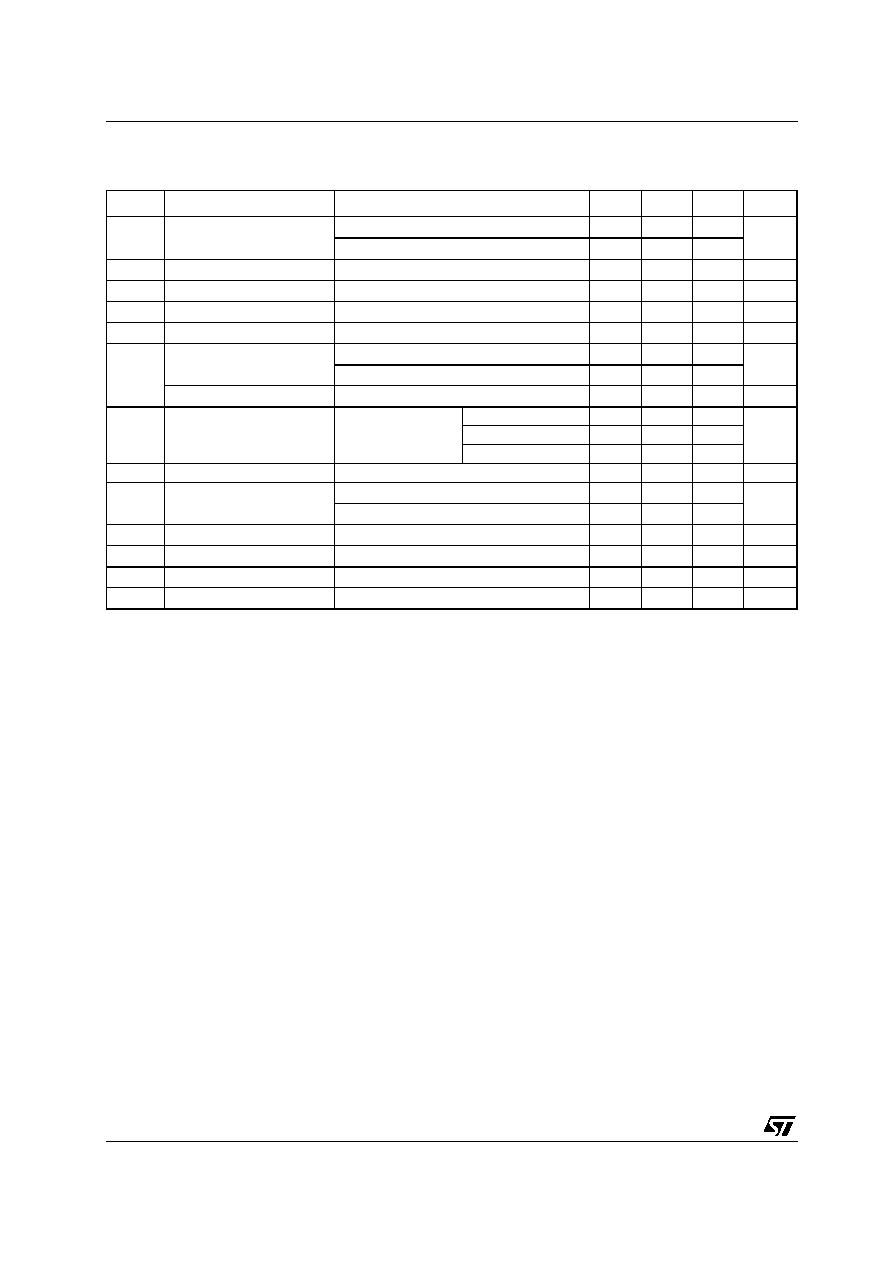L4931 SERIES
20/29
ELECTRICAL CHARACTERISTICS OF L4931Cxx120 (refer to the test circuits, T
J
= 25°C,
C
I
= 0.1 µF, C
O
= 2.2 µF unless otherwise specified).
(*) For SO-8 package the maximum limit of load regulation and dropout is increased by 20 mV.
Symbol
Parameter
Test Conditions
Min.
Typ.
Max.
Unit
V
O
Output Voltage
I
O
= 5 mA
V
I
= 14 V
11.76
12
12.24
V
I
O
= 5 mA
V
I
= 14 V
T
A
=-25 to 85°C
11.52
12.48
V
I
Operating Input Voltage
I
O
= 250 mA
20
V
I
out
Output Current Limit
300
mA
V
O
Line Regulation
V
I
= 12.9 to 20 V
I
O
= 0.5 mA
4
24
mV
V
O
(*)
Load Regulation
V
I
= 13.1 V
I
O
= 0.5 to 250 mA
3
18
mV
I
d
Quiescent Current
ON MODE
V
I
= 13.1 to 20 V
I
O
= 0 mA
0.8
1.6
mA
V
I
= 13.1 to 20 V
I
O
= 250 mA
4.5
7
OFF MODE
V
I
= 6 V
90
180
µA
SVR
Supply Voltage Rejection
I
O
= 5 mA
V
I
= 14.1 ± 1 V
f = 120 Hz
64
dB
f = 1 KHz
61
f = 10 KHz
55
eN
Output Noise Voltage
B =10 Hz to 100 KHz
50
µ
V
V
d
(*)
Dropout Voltage
I
O
= 250 mA
0.4
0.6
V
I
O
= 250 mA
T
J
= -40 to 125°C
0.8
V
IL
Control Input Logic Low
T
A
= -40 to 125°C
0.8
V
V
IH
Control Input Logic High
T
A
= -40 to 125°C
2
V
I
I
Control Input Current
V
I
= 6 V,
V
C
= 6 V
10
µA
C
O
Output Bypass Capacitance ESR = 0.1 to 10
I
O
= 0 to 250 mA
2
10
µF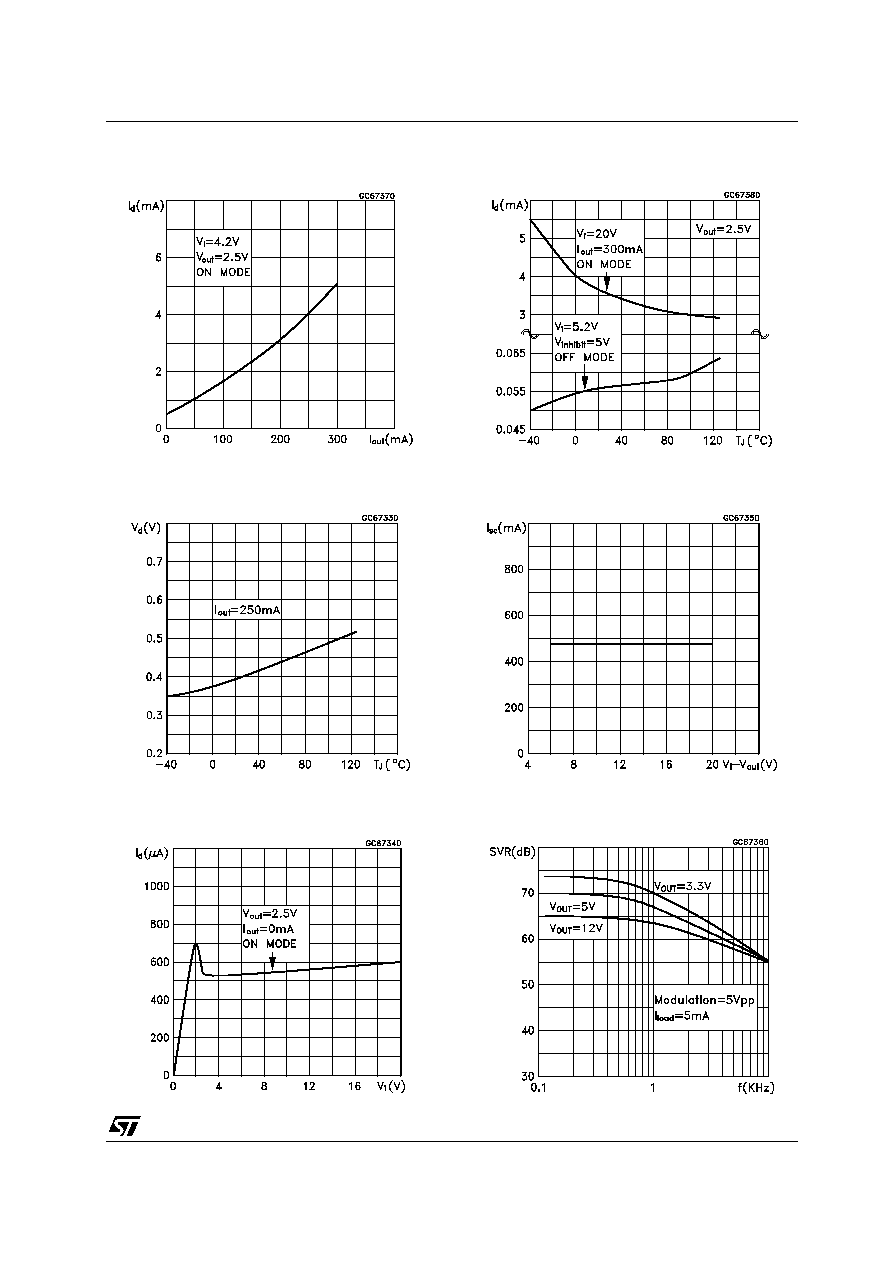L4931 SERIES
21/29
Figure 1 : Supply Current vs Output Current
Figure 2 : Dropout Voltage vs Temperature
Figure 3 : Supply Current vs Input Voltage
Figure 4 : Supply Current vs Temperature
Figure 5 : Short Circuit Current vs Dropout
Voltage
Figure 6 : S.V.R. vs Input Voltage Signal
Frequency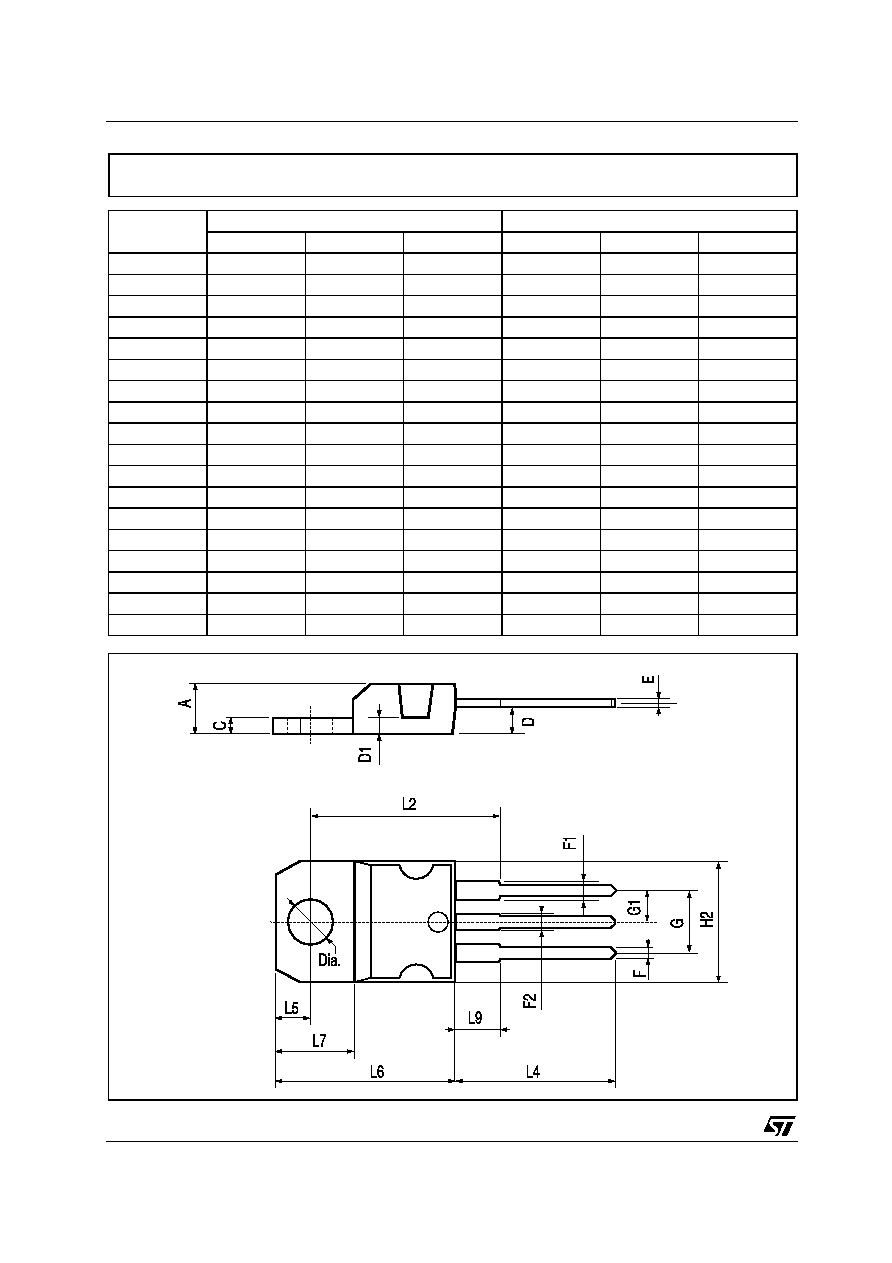L4931 SERIES
22/29
DIM.
mm.
inch
MIN.
TYP
MAX.
MIN.
TYP.
MAX.
A
4.40
4.60
0.173
0.181
C
1.23
1.32
0.048
0.051
D
2.40
2.72
0.094
0.107
D1
1.27
0.050
E
0.49
0.70
0.019
0.027
F
0.61
0.88
0.024
0.034
F1
1.14
1.70
0.044
0.067
F2
1.14
1.70
0.044
0.067
G
4.95
5.15
0.194
0.203
G1
2.4
2.7
0.094
0.106
H2
10.0
10.40
0.393
0.409
L2
16.4
0.645
L4
13.0
14.0
0.511
0.551
L5
2.65
2.95
0.104
0.116
L6
15.25
15.75
0.600
0.620
L7
6.2
6.6
0.244
0.260
L9
3.5
3.93
0.137
0.154
DIA.
3.75
3.85
0.147
0.151
TO-220 MECHANICAL DATA
P011C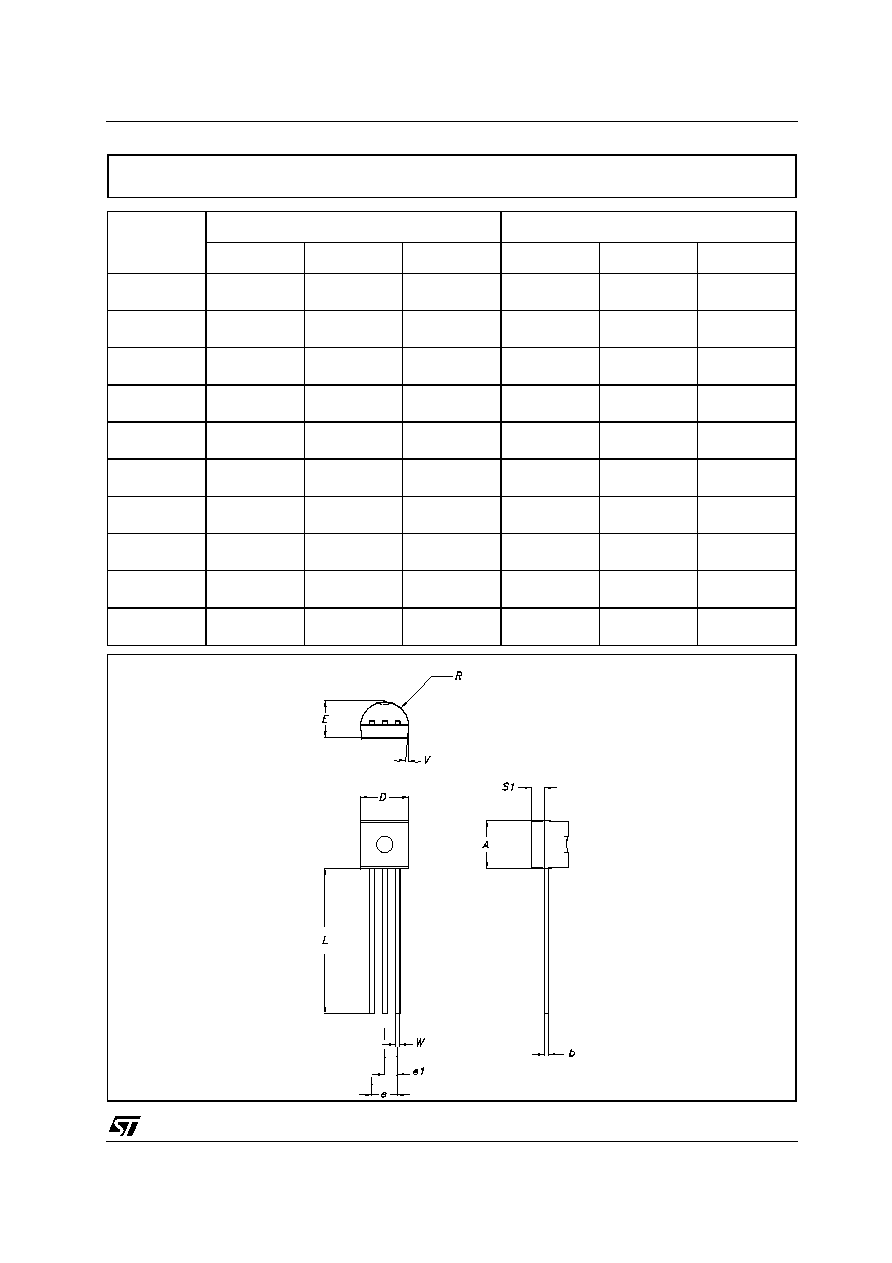L4931 SERIES
23/29
DIM.
mm.
mils
MIN.
TYP
MAX.
MIN.
TYP.
MAX.
A
4.32
4.95
170.1
194.9
b
0.36
0.51
14.2
20.1
D
4.45
4.95
175.2
194.9
E
3.30
3.94
129.9
155.1
e
2.41
2.67
94.9
105.1
e1
1.14
1.40
44.9
55.1
L
12.7
15.49
500.0
609.8
R
2.16
2.41
85.0
94.9
S1
0.92
1.52
36.2
59.8
W
0.41
0.56
16.1
22.0
TO-92 MECHANICA DATA
0102782/C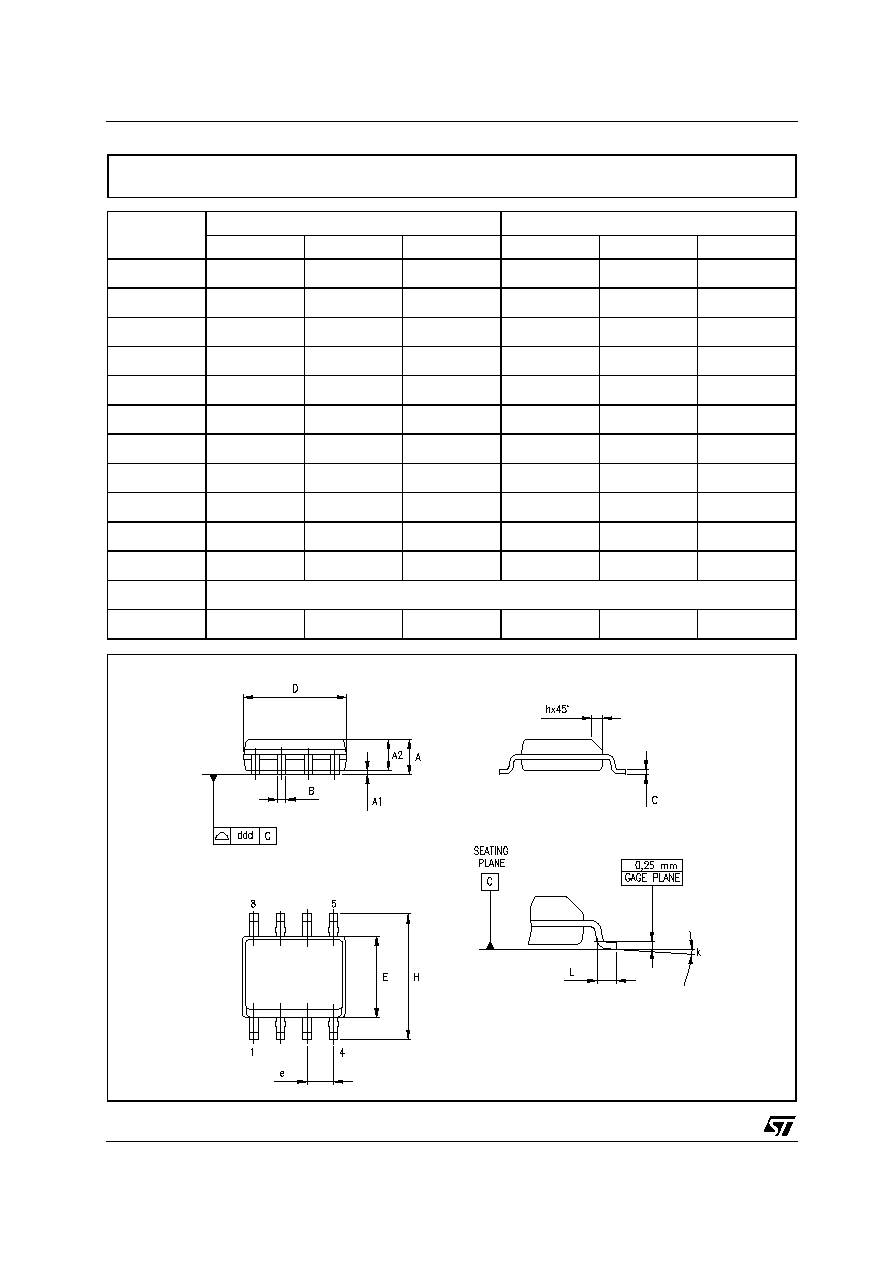L4931 SERIES
24/29
DIM.
mm.
inch
MIN.
TYP
MAX.
MIN.
TYP.
MAX.
A
1.35
1.75
0.053
0.069
A1
0.10
0.25
0.04
0.010
A2
1.10
1.65
0.043
0.065
B
0.33
0.51
0.013
0.020
C
0.19
0.25
0.007
0.010
D
4.80
5.00
0.189
0.197
E
3.80
4.00
0.150
0.157
e
1.27
0.050
H
5.80
6.20
0.228
0.244
h
0.25
0.50
0.010
0.020
L
0.40
1.27
0.016
0.050
k
° (max.)
ddd
0.1
0.04
SO-8 MECHANICAL DATA
0016023/C
8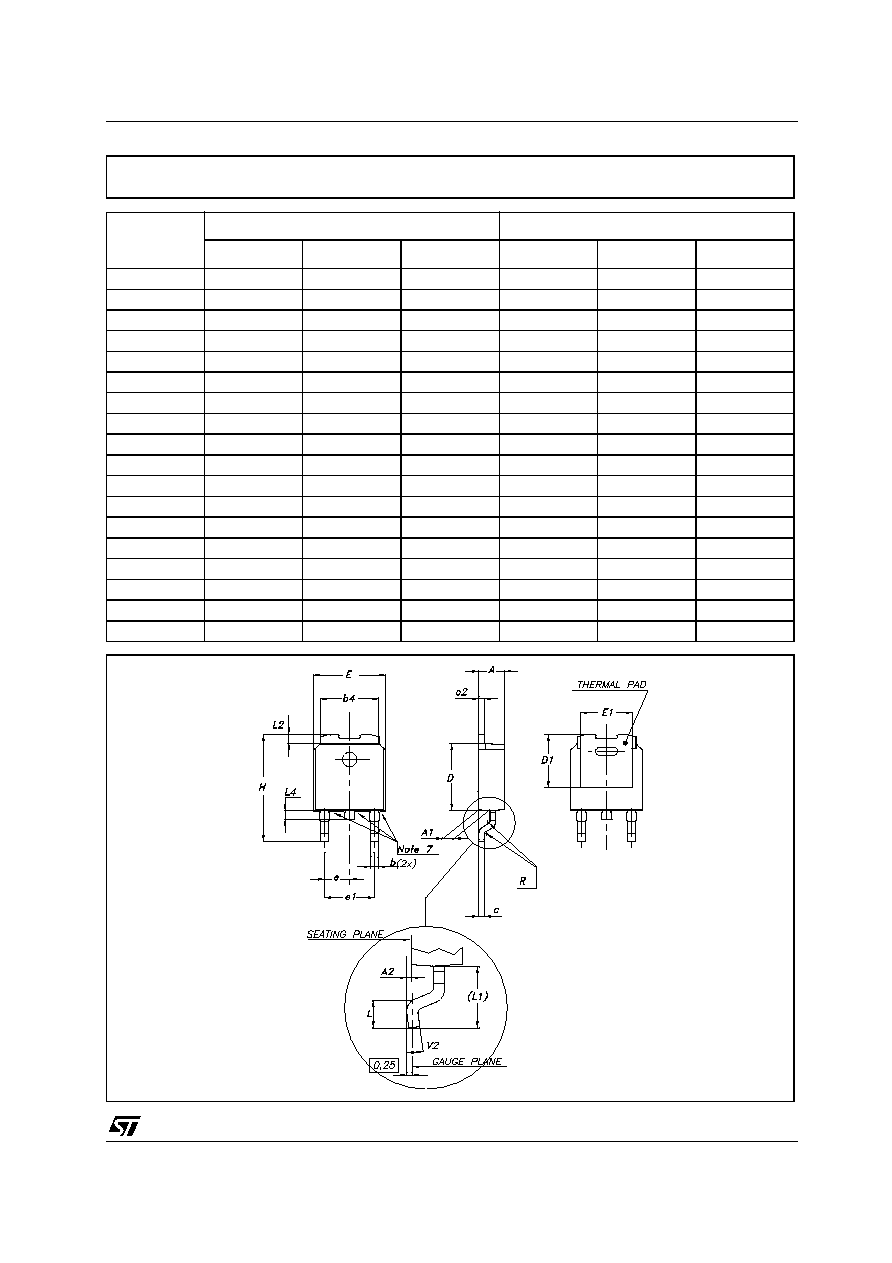L4931 SERIES
25/29
DIM.
mm.
inch
MIN.
TYP
MAX.
MIN.
TYP.
MAX.
A
2.2
2.4
0.086
0.094
A1
0.9
1.1
0.035
0.043
A2
0.03
0.23
0.001
0.009
B
0.64
0.9
0.025
0.035
B2
5.2
5.4
0.204
0.212
C
0.45
0.6
0.017
0.023
C2
0.48
0.6
0.019
0.023
D
6
6.2
0.236
0.244
D1
5.1
0.200
E
6.4
6.6
0.252
0.260
E1
4.7
0.185
e
2.28
0.090
e1
4.4
4.6
0.173
0.181
H
9.35
10.1
0.368
0.397
L
1
0.039
(L1)
2.8
0.110
L2
0.8
0.031
L4
0.6
1
0.023
0.039
DPAK MECHANICAL DATA
0068772-F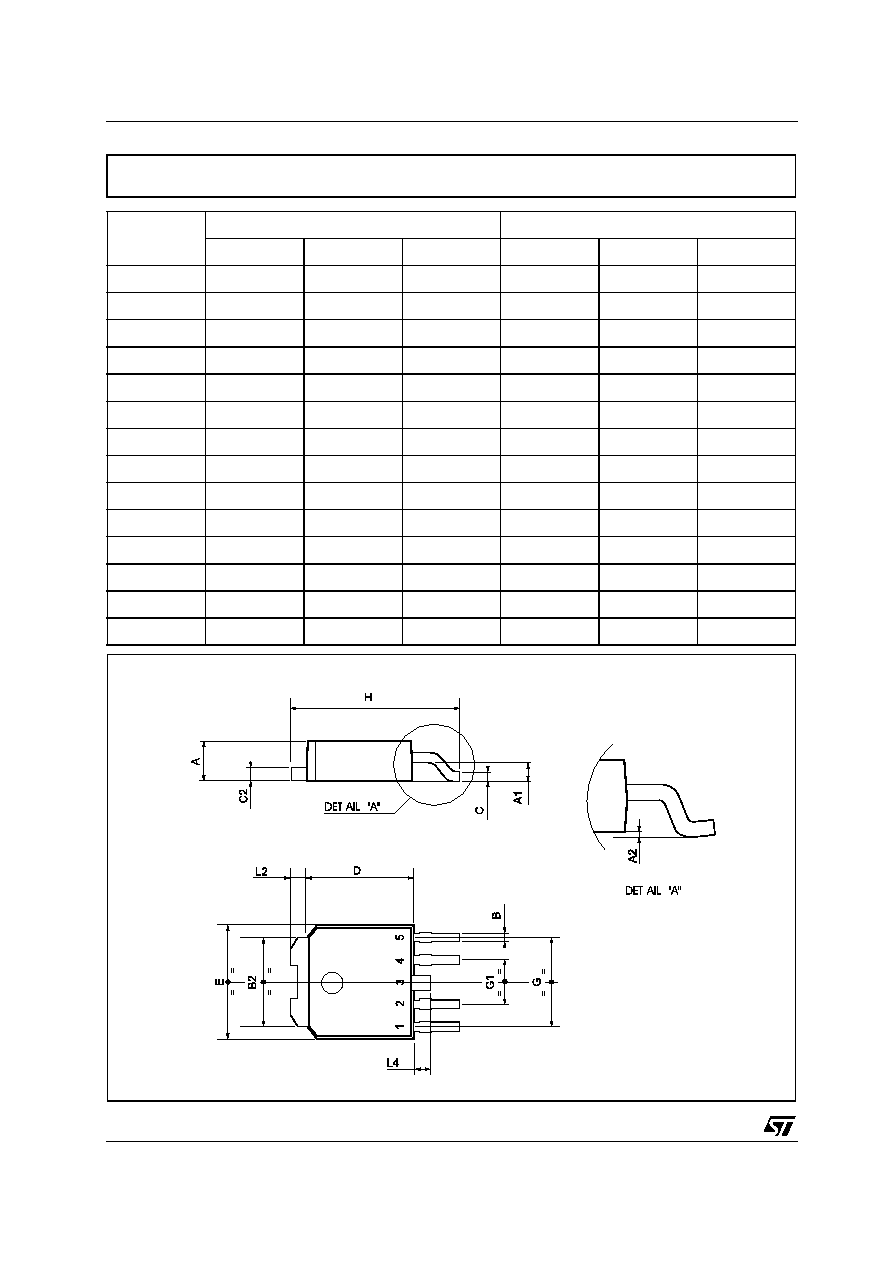L4931 SERIES
26/29
DIM.
mm.
inch
MIN.
TYP
MAX.
MIN.
TYP.
MAX.
A
2.2
2.4
0.086
0.094
A1
0.9
1.1
0.035
0.043
A2
0.03
0.23
0.001
0.009
B
0.4
0.6
0.015
0.023
B2
5.2
5.4
0.204
0.212
C
0.45
0.6
0.017
0.023
C2
0.48
0.6
0.019
0.023
D
6
6.2
0.236
0.244
E
6.4
6.6
0.252
0.260
G
4.9
5.25
0.193
0.206
G1
2.38
2.7
0.093
0.106
H
9.35
10.1
0.368
0.397
L2
0.8
0.031
L4
0.6
1
0.023
0.039
PPAK MECHANICAL DATA
0078180-B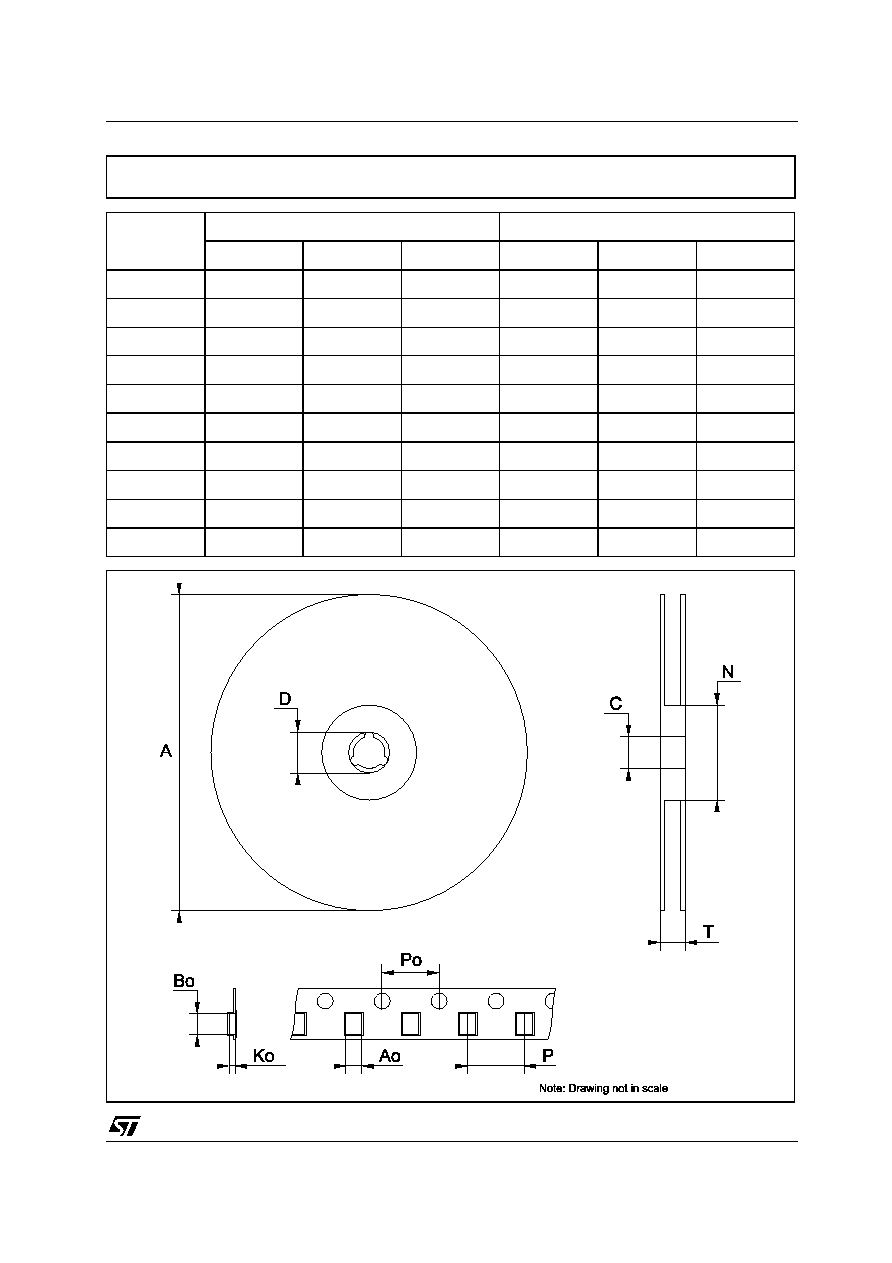L4931 SERIES
27/29
DIM.
mm.
inch
MIN.
TYP
MAX.
MIN.
TYP.
MAX.
A
330
12.992
C
12.8
13.0
13.2
0.504
0.512
0.519
D
20.2
0.795
N
60
2.362
T
22.4
0.882
Ao
6.80
6.90
7.00
0.268
0.272
0.2.76
Bo
10.40
10.50
10.60
0.409
0.413
0.417
Ko
2.55
2.65
2.75
0.100
0.104
0.105
Po
3.9
4.0
4.1
0.153
0.157
0.161
P
7.9
8.0
8.1
0.311
0.315
0.319
Tape & Reel DPAK-PPAK MECHANICAL DATA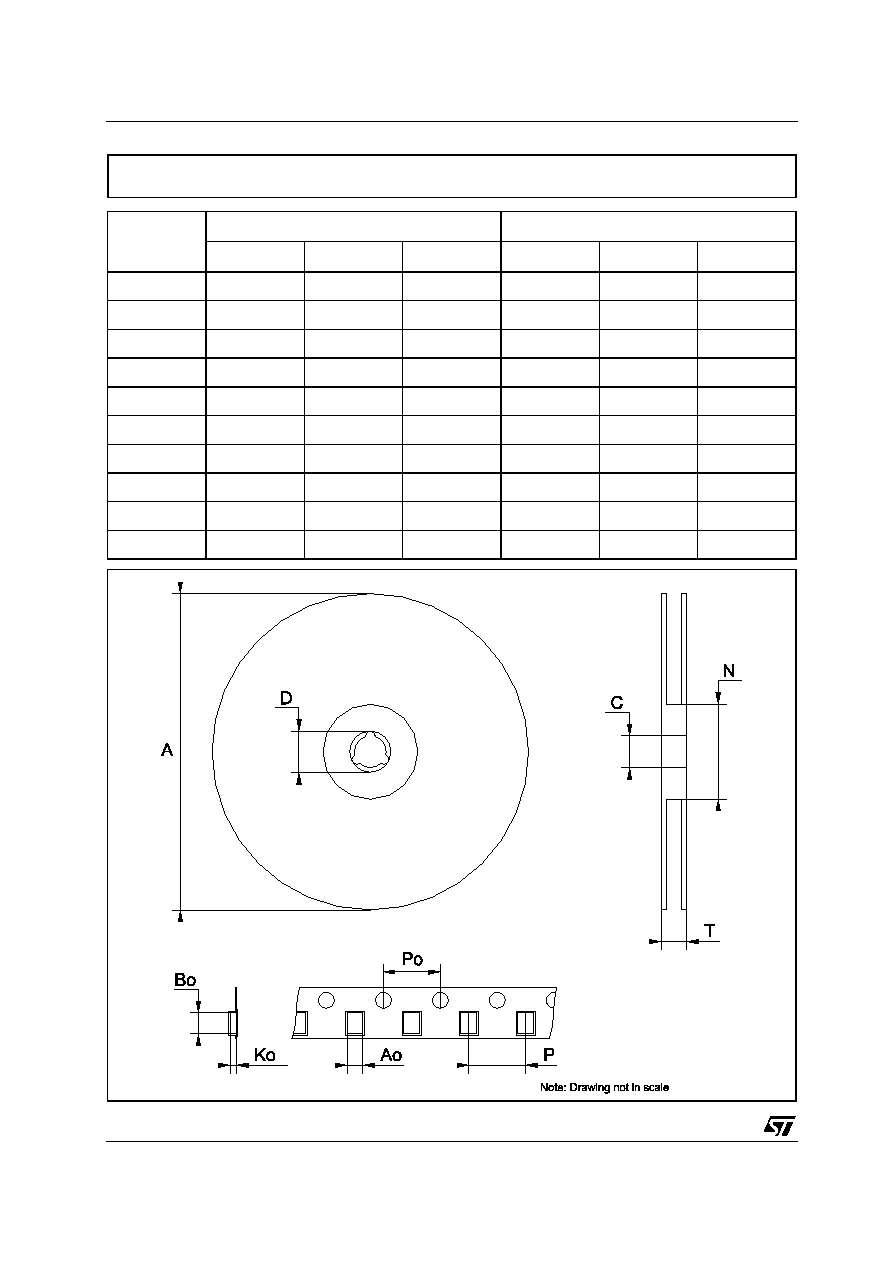L4931 SERIES
28/29
DIM.
mm.
inch
MIN.
TYP
MAX.
MIN.
TYP.
MAX.
A
330
12.992
C
12.8
13.2
0.504
0.519
D
20.2
0.795
N
60
2.362
T
22.4
0.882
Ao
8.1
8.5
0.319
0.335
Bo
5.5
5.9
0.216
0.232
Ko
2.1
2.3
0.082
0.090
Po
3.9
4.1
0.153
0.161
P
7.9
8.1
0.311
0.319
Tape & Reel SO-8 MECHANICAL DATA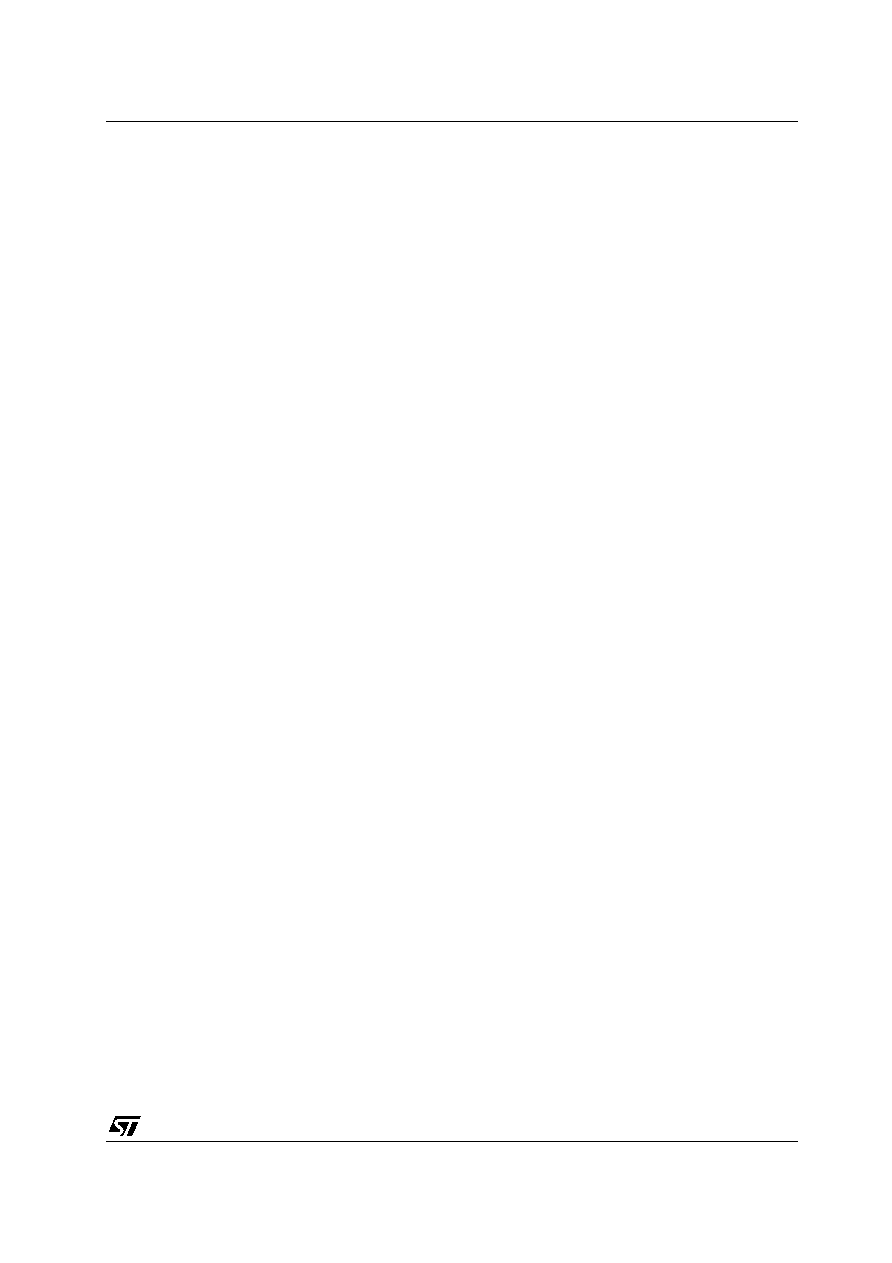L4931 SERIES
29/29
Information furnished is believed to be accurate and reliable. However, STMicroelectronics assumes no responsibility for the
consequences of use of such information nor for any infringement of patents or other rights of third parties which may result from
its use. No license is granted by implication or otherwise under any patent or patent rights of STMicroelectronics. Specifications
mentioned in this publication are subject to change without notice. This publication supersedes and replaces all information
previously supplied. STMicroelectronics products are not authorized for use as critical components in life support devices or
systems without express written approval of STMicroelectronics.
The ST logo is a registered trademark of STMicroelectronics
All other names are the property of their respective owners
© 2004 STMicroelectronics - All Rights Reserved
STMicroelectronics GROUP OF COMPANIES
Australia - Belgium - Brazil - Canada - China - Czech Republic - Finland - France - Germany - Hong Kong - India - Israel - Italy - Japan -
Malaysia - Malta - Morocco - Singapore - Spain - Sweden - Switzerland - United Kingdom - United States.
http://www.st.com© 2019 • ICSheet• Contact form• Main page